# Pivot table

Modified on Fri, 29 Sep 2023 at 04:41 PM

# Cell/sample selection and metadata quantification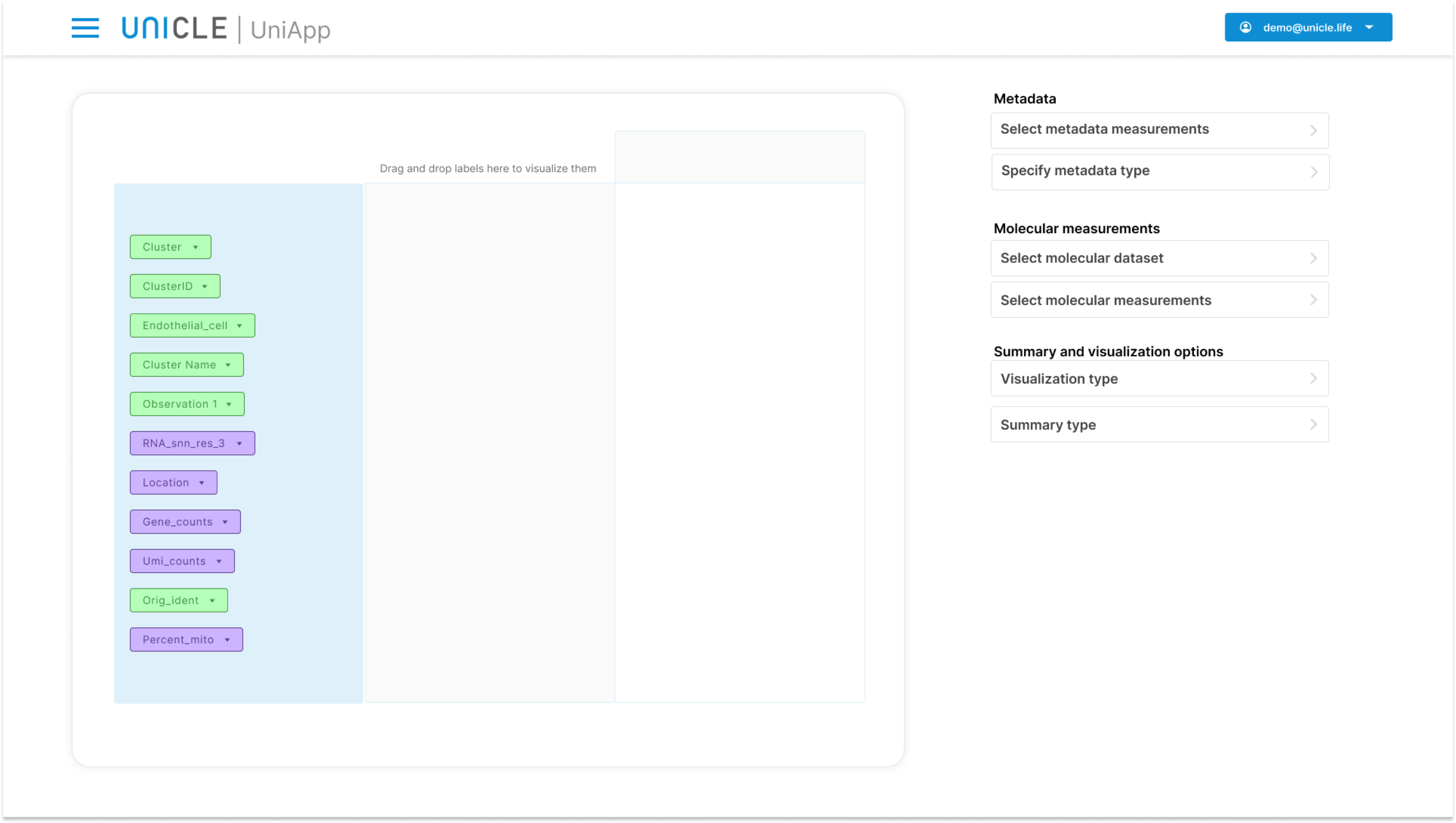The UniApp provides flexible data analysis by allowing to subset your data in a virtually unlimited manner. This offers great flexibility in your analysis and experimental design, and can be accomplished in the Pivot table module.

# 1 Algorithm settings

## 1.1 Creating a new plot

To begin, you should assign a name, write a description for your plot and choose "Pivot table" as your analysis algorithm. You can find these on the Create Plot field. Then, you should choose "Pivot table" from the Choose algorithm to run your analysis dropdown menu. Once you input all desired information, you can click on Select algorithm button to finish this stage.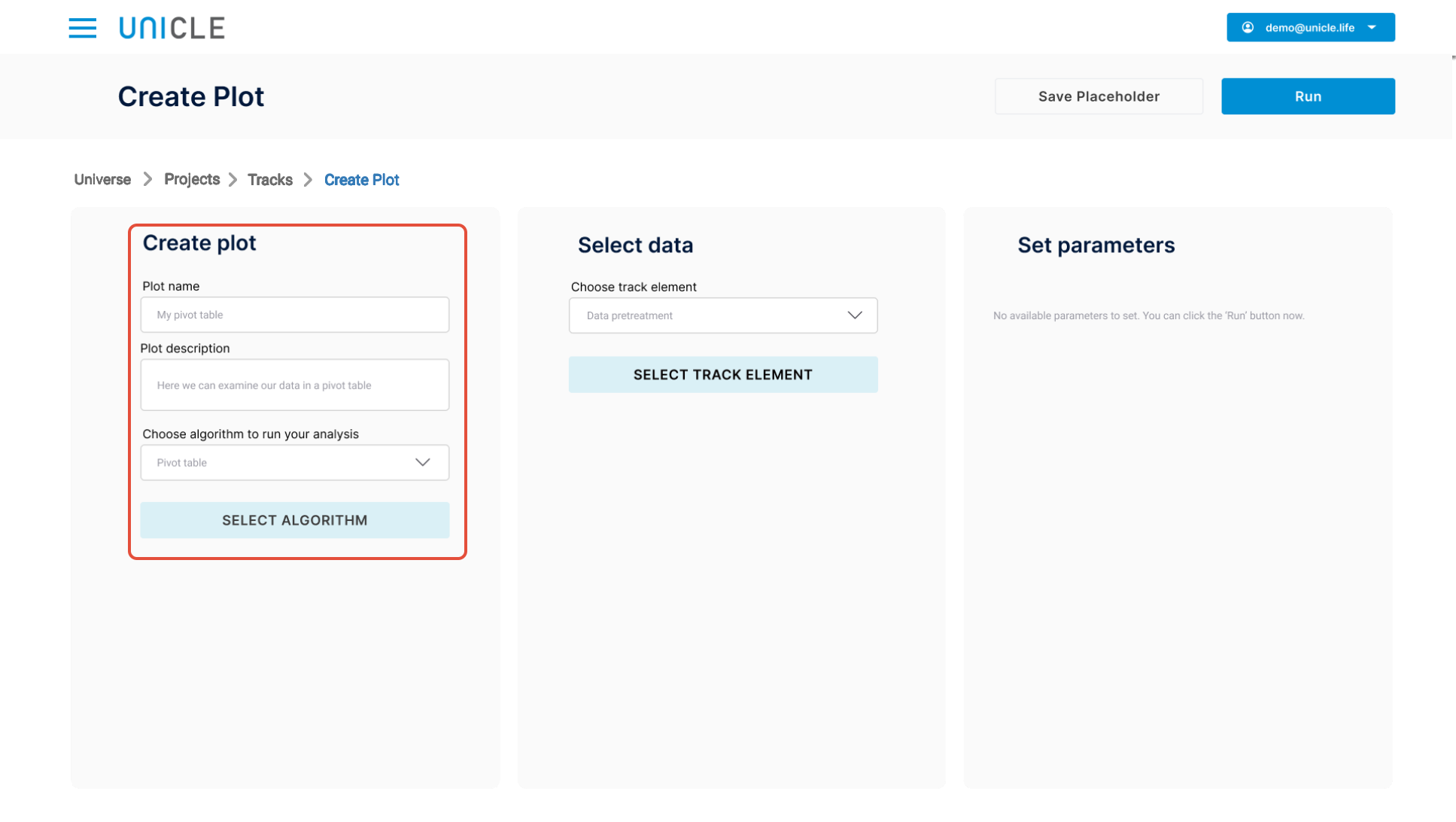## 1.2 Select data

In this step, you need to present the right data to UniApp to be processed. By clicking on Choose track element dropdown menu, you can select the input data.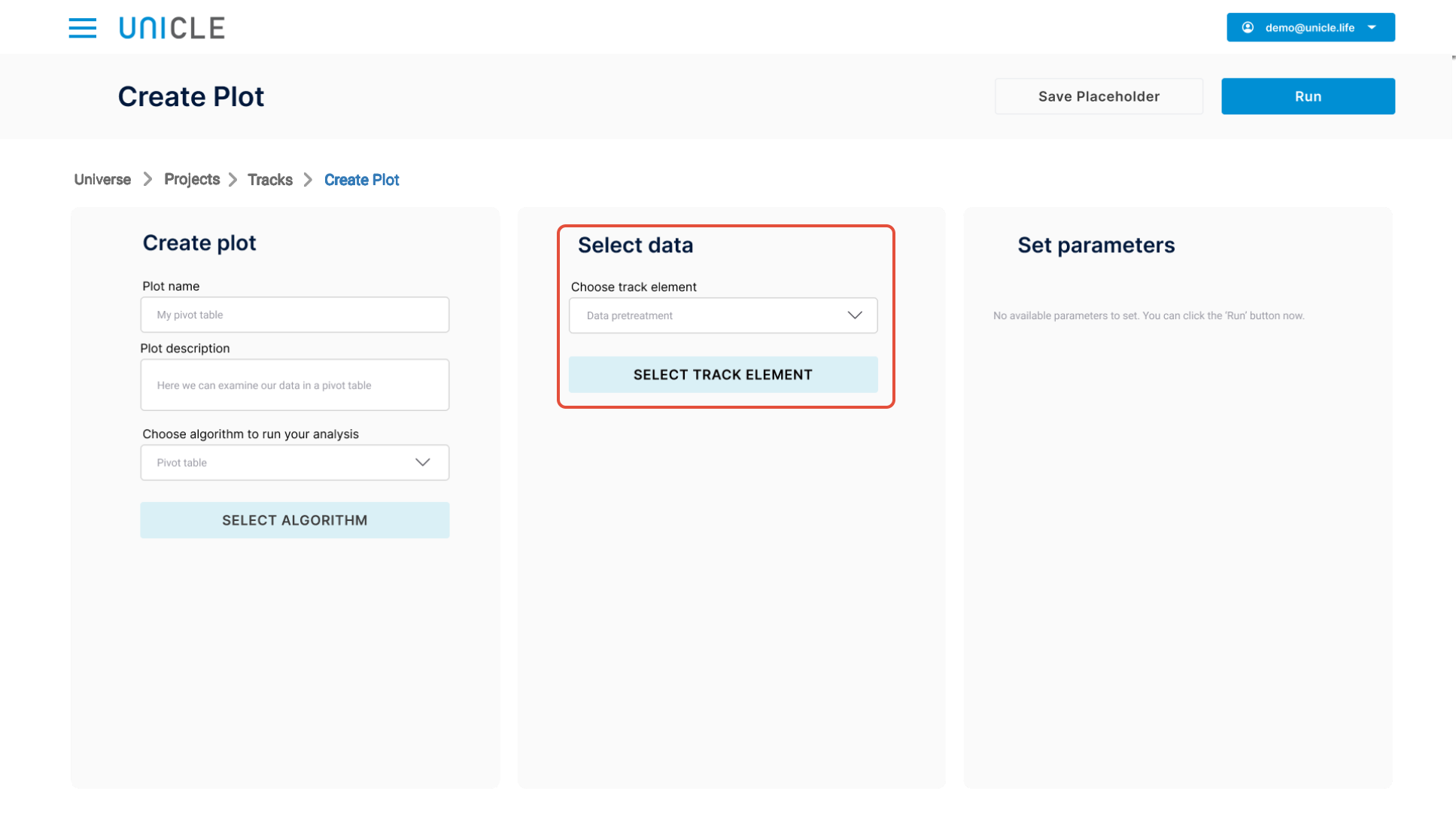## 1.3 Selecting parameters

The spatial expression visualization algorithm does not have any available parameters at the moment. You can proceed to run the analysis by clicking the Run button.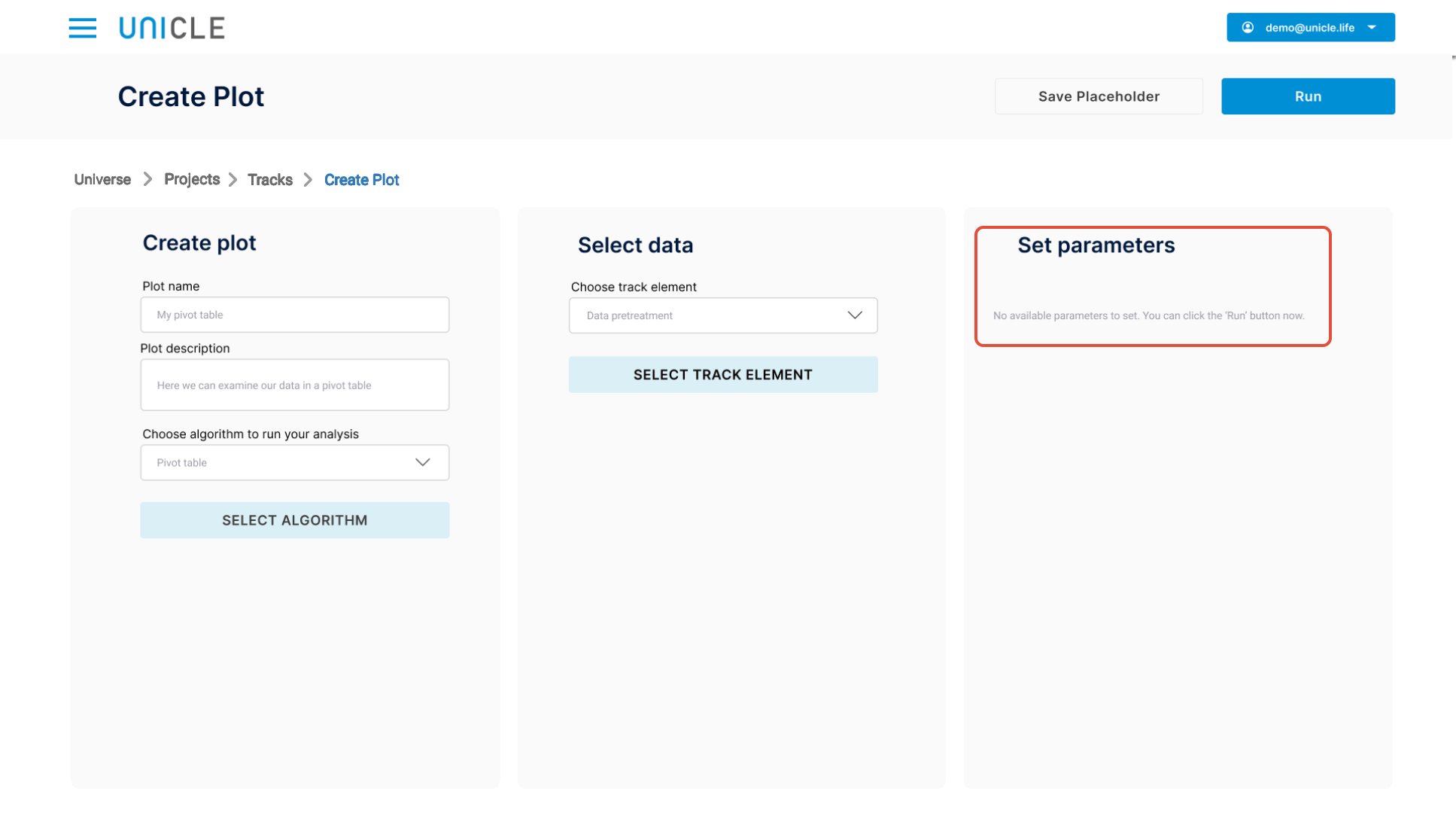# 2. Spatial expression visualization interactive plot page

In the pivot table interactive plot page you can interactively explore your data.

## 2.1 Pivot table layout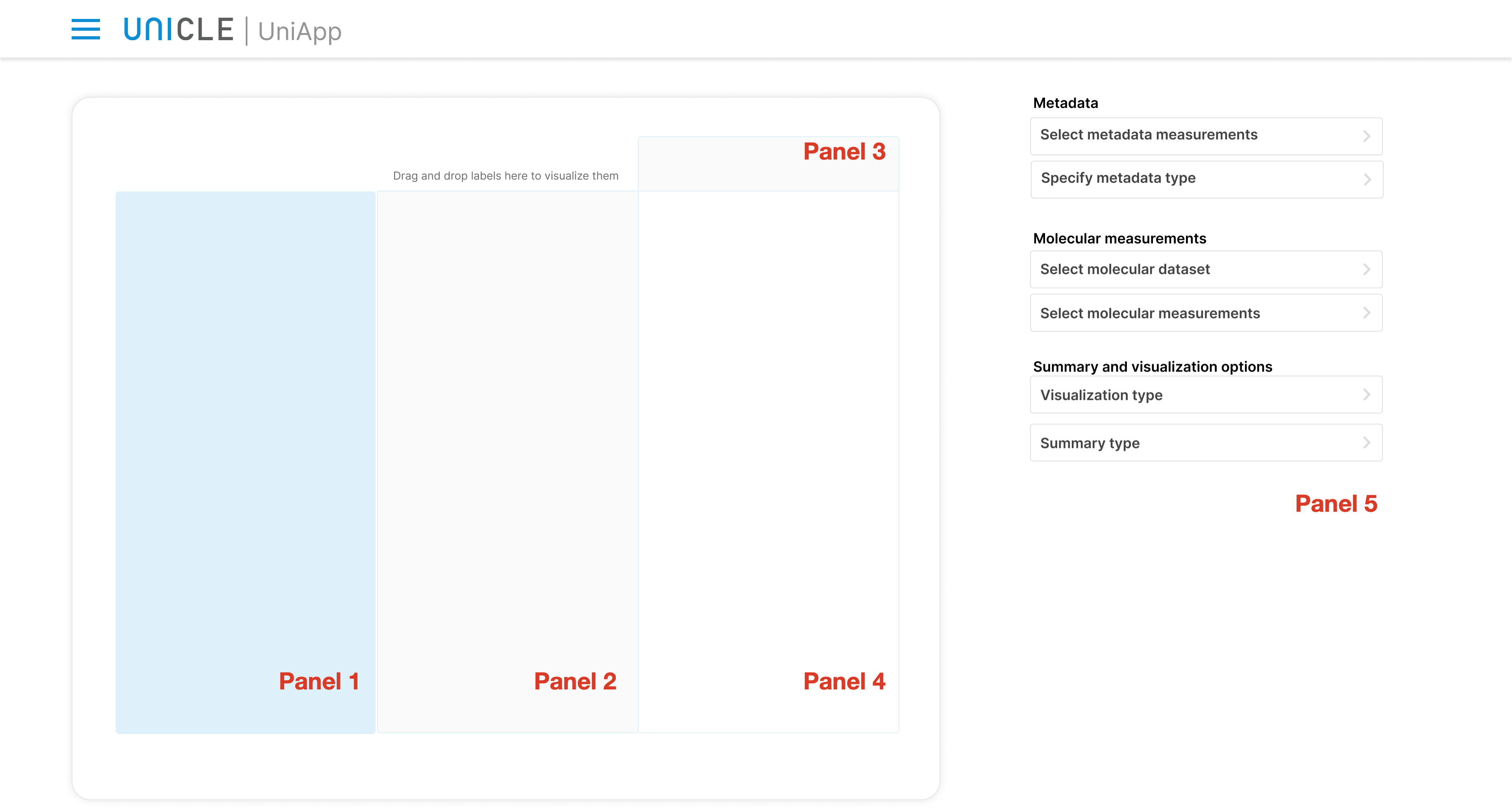The pivot table is partitioned in six panels:

• Panel 1 lists all metadata variables. From here you can drag and drop  variables to Panel 2 and Panel 3 to determine which variables make up the rows and columns of the pivot table, respectively. By default, this panel is empty until the user selects the measurements to work with (see below).
• Panel 2 is the area where you can drag and drop metadata variables to be summarized and visualized in Panel 4. This will correspond to the y-axis of the plots visualized in Panel 4.
• Panel 3 is the second area where you can drag and drop metadata variables to further details the plots and tables displayed in Panel 4.
• Panel 4 displays the resulting pivot table or visualization. By default is empy until the user configure a visualization.
• Panel 5 presents different drop-down menus for further customize the pivot table. Some are available by default (see list below), other appears depending on the pivot table configuration
• "Select metadata measurements" allows to decide which metadata variables are displayed in Panel 1.
• "Specify metadata type": here the users can specify whether the selected metadata measurements are categorical or numerical
• "Select molecular dataset": the same molecular dataset can be present with different preprocessing: normalized, scaled, log-transformed, etc. This menu allows to decide which one to use.
• "Select molecular measurements" allows to select from the chosen molecular dataset one or more genes / metabolites / proteins (depending on the nature of your omics dataset). The seleted variable available for exploration with the pivot table.
• "Visualization type" specifies how the data are visualized in the Pivot Table (Panel 4). Particularly, it allows to switch between a tabular visualization of the data and different graphical representations (plots).
• "Summary type"  defines how your data should be aggregated. For example, Here you can decide to visualize the average values rather than the maximum values for a continuous variable.

## 2.2 Selecting measurements

Each project can involve a large number of measurements (a.k.a. variables), recorded either as metadata (e.g., phenotype information) or molecular measurments (e.g., gene expression levels, protein abundance levels). The user is first asked to select the subset of variables that should be analyzed within the pivot table. This can be done through the buttons in Panel 5.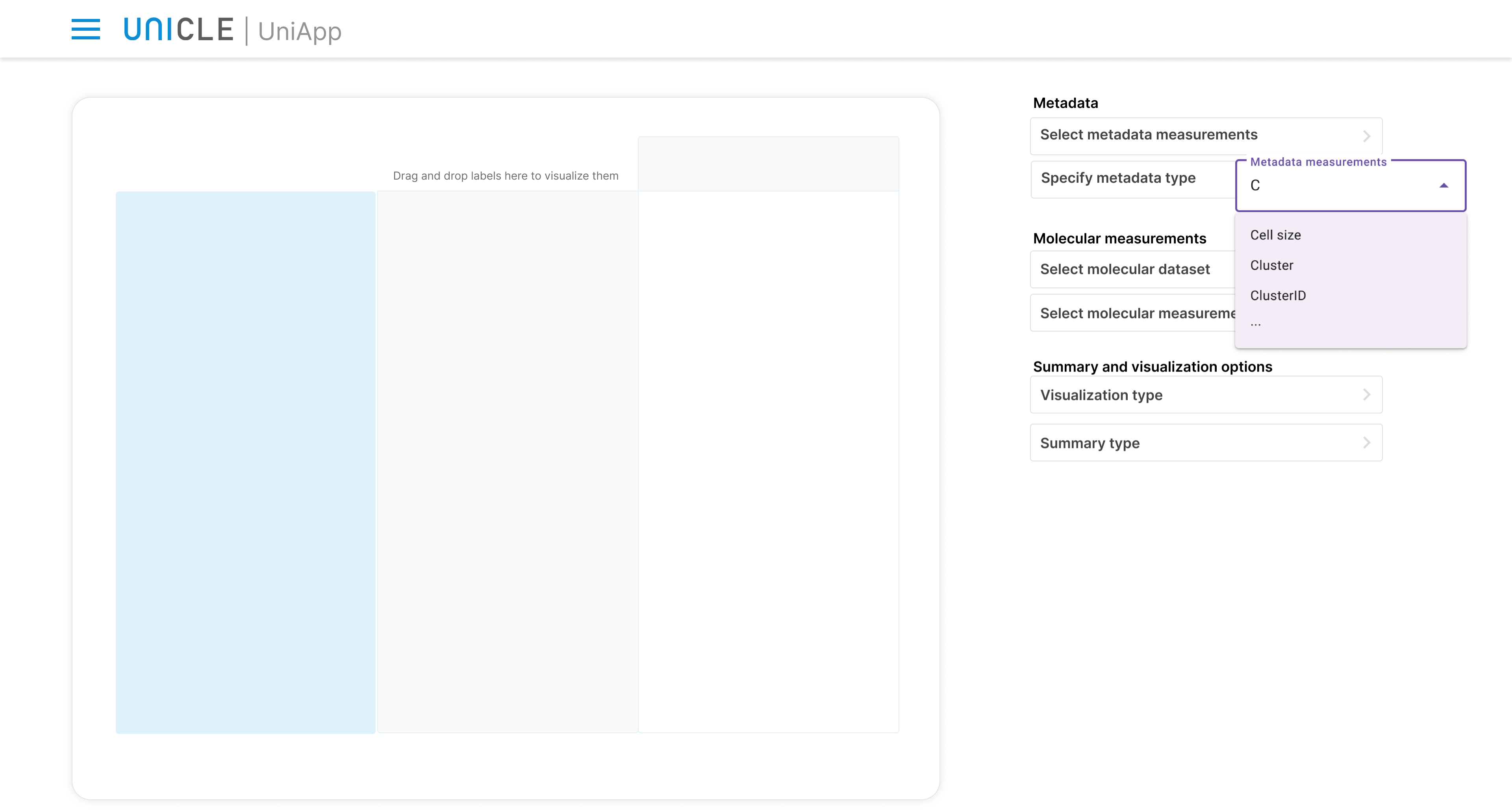The Select metadata measurements button provides a searchable menu where the user can select the metadata variables to be listed in Panel 1 and being included in the analysis.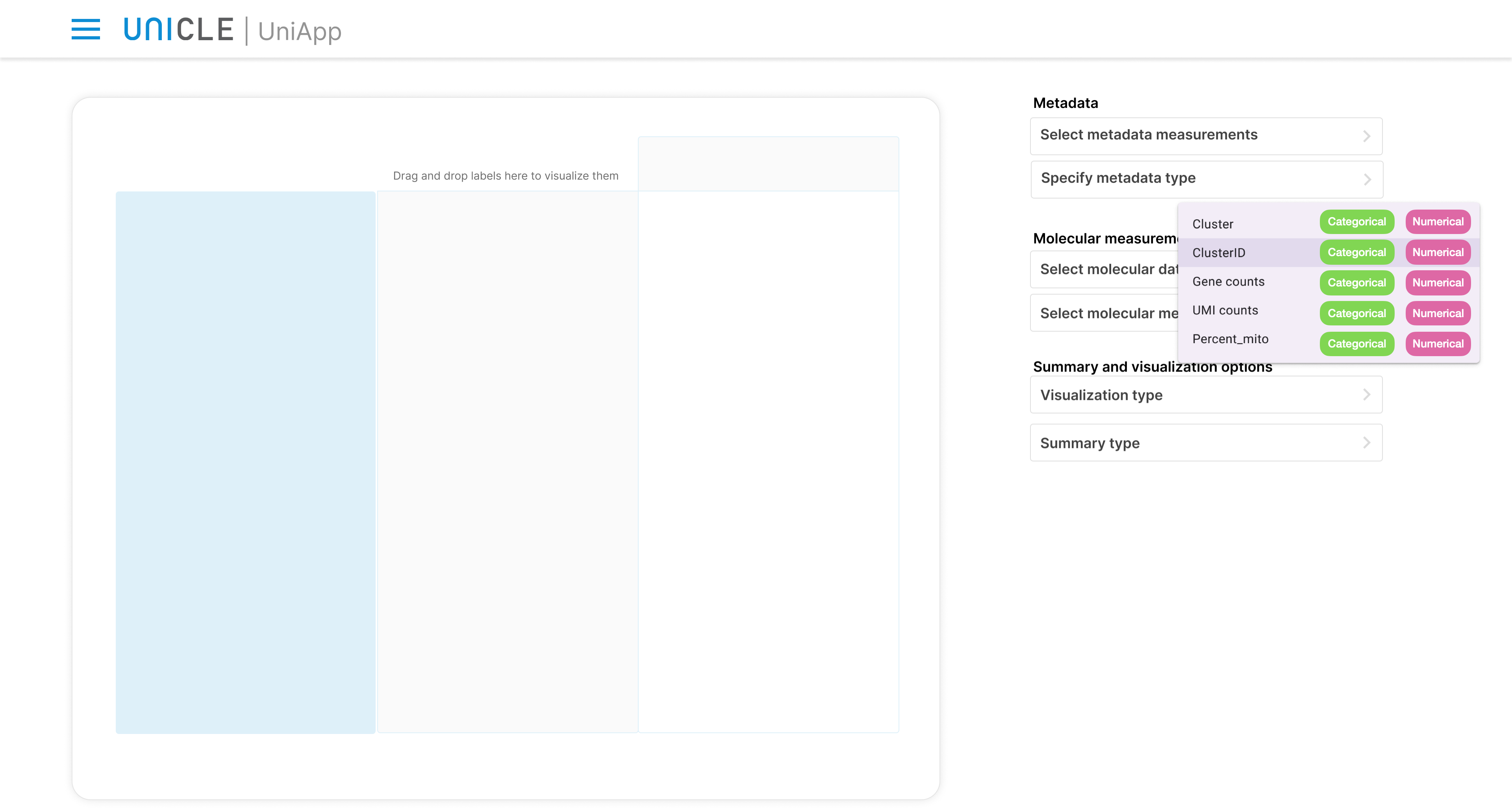The Specify metadata type button instead allows the user to specify whether each selected metadata variable is numerical or categorical (see next subsection for more details on this topic).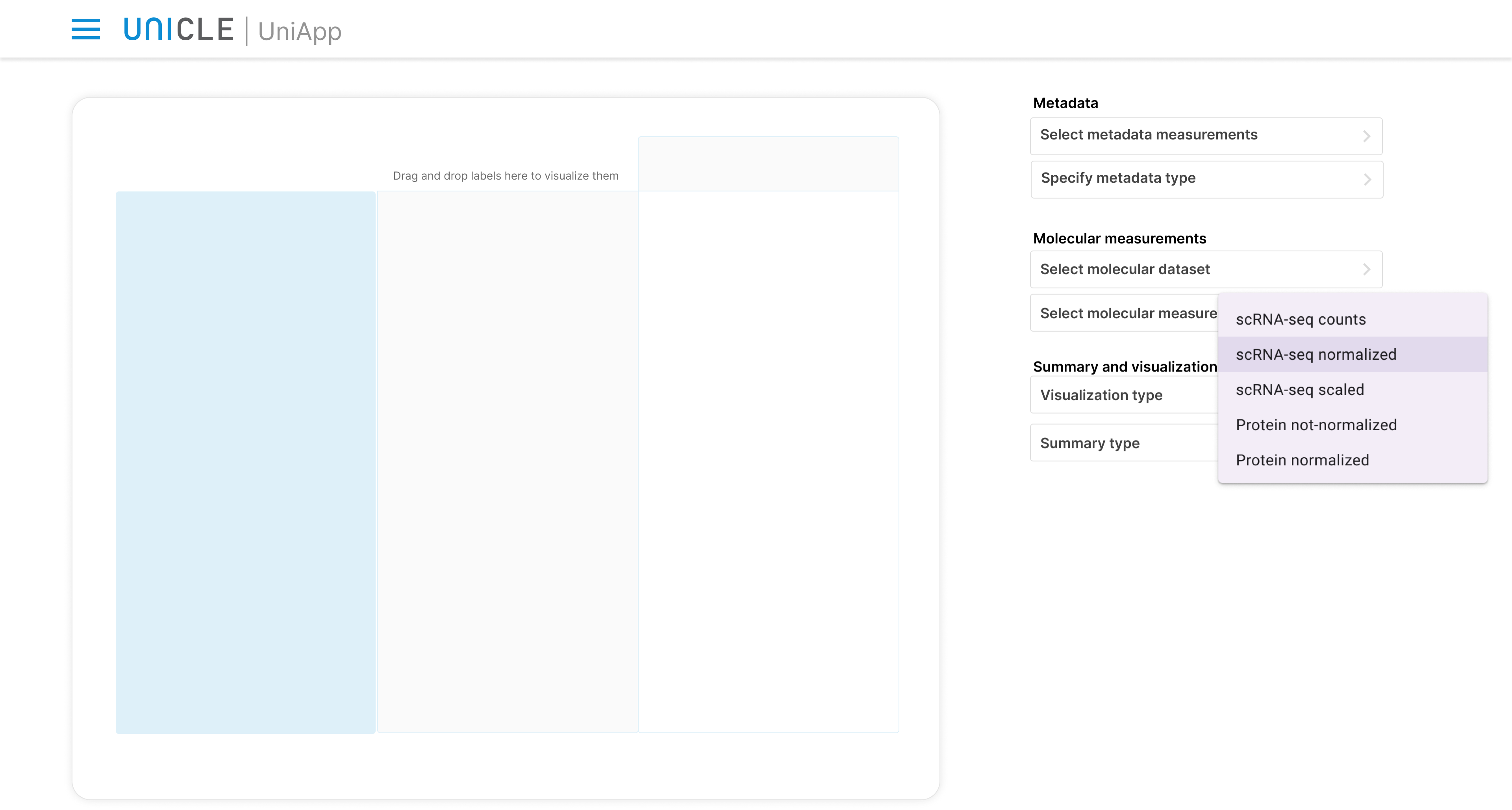The next button, Select molecular dataset, can be used for selecting a specific molecular dataset from which extraxcting molecular measurements to analyze. If several version of the same dataset (e.g, normalized, scaled, etc.) are present, they will all appear in this menu.With the Select molecular measurements button, the user can finally spaecify which molecular variables should be listed in Panel 1.

### 2.2.1 Variables types

Metadata can be represented by different types of variables. The Pivot Table will present different visualizations depending pn which types of variables are selected. UniApp distinguishes between two main types:

1. Numerical variables: measurements like age, height, number of cells are all numerical variables. Further subcategories are possible as well:
1. Count values: quantities like number of cells or number of patients are inherently discrete, meaning they can only assume values that are integer: 0, 1, 2, etc.
2. Continuous values: these variables can assume any real numerical values, e.g., 3.8, 4.1, etc.
2. Categorical variables: these measurements contain categories like colors or locations that cannot usually be described as numbers. These variables can be further classified as:
1. Binary: containing only two values, e.g., Yes or No, True or False.
2. Nominal: they contain more than two values, e.g., red, yellow, blue, or east, west, north and south
3. Ordinal: these variables have a few number of categories (less than 10), and an order can be established among the catergories, for example short, medium, tall.

Numerical variables are marked violet, while categorical variables in green.

## 2.3 Selecting subsets of cells

To subset you data, in the bottom left field where all metadata variables (i.e. columns) are listed, click on the downward pointing arrow to right of a metadata variable. The subsetting mechanism is different for numerical or categorical variables.

Selecting cells in the Data pretreatment module will perform a "hard" subset. This means that only the cells in the cell subset used in data pretreatment will be available in the downstream analyses.

### 2.3.1 Selecting subsets of cells on the basis of a categorical variable

If you select a categorical variable, then you perform the subsetting by selecting the categories to keep / discard. For example, in the metadata "ClusterID" we can deselect all cells corresponding to clusters 6 and 7. This means that these cells will not be retained for the following visualizations / analyses. Click on the Apply button to save your selection.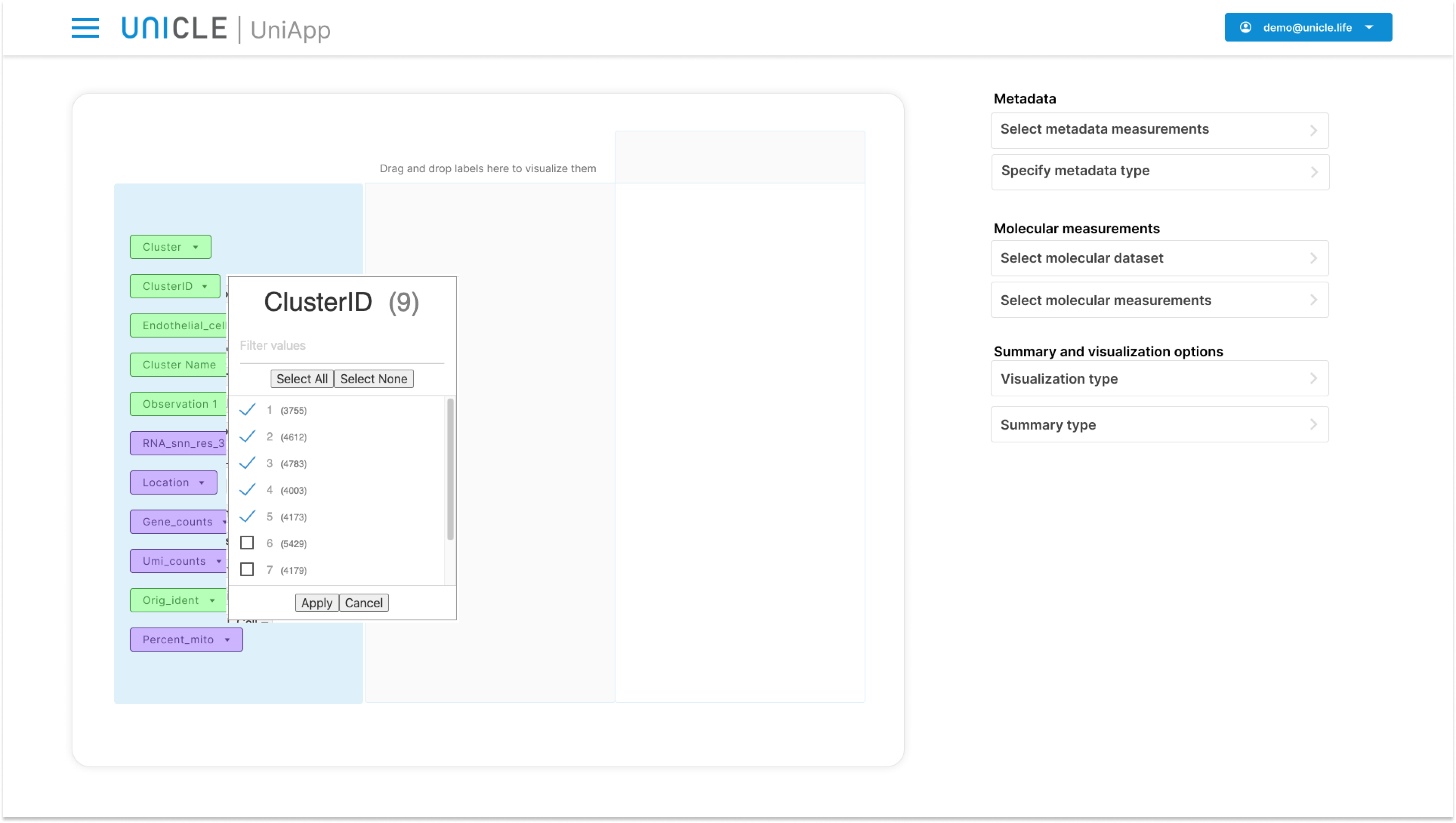### 2.3.1 Selecting subsets of cells on the basis of a numerical variable

If you select a numerical variable, then you must specify an interval of suitable values that corresponds to cells / samples / observations that must be retained.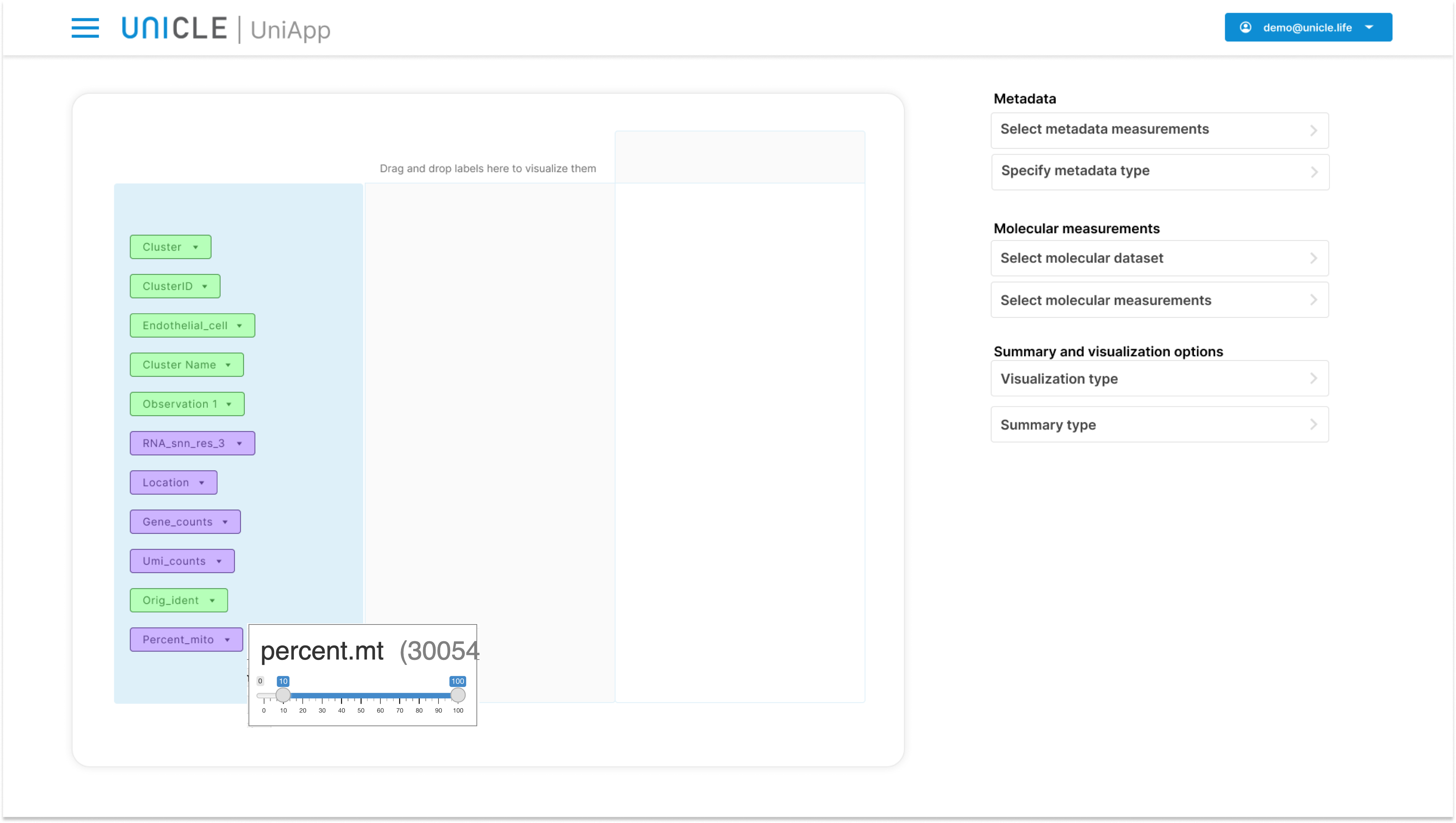Subsetting can be performed on multiple different variables at same time. For example, we may select on the basis of both "ClusterID" and "percent_mito".

## 2.4 Visualizing single variables

In order to visualize the properties of a numerical variable you need to drag the variable from Panel 1 to Panel 2 or, equivalently, Panel 3.

`Depending on whether the selected variable is categorical or numerical, the type of information that can be visualized changes. `

Particularly, the options that are available in the two dropdown menus "Visualization types" and "Summary Type" of Panel 5 will change depending on the type of seleted variables.

### 2.4.1 Visualizing a single numerical variable

As soon as a single numerical variable is dropped in Panel 2 (or Panel 3), the following visualization types will become available in the dropdown menu of Panel 5:

1. Statistics
2. Histogram
3. Density plot

The "Summary typedropdown menu in Panel 2 will be disabled, since  for numerical variables we only visualize their original distribution.

The following plot presents the "Statistics: summary" visualization for single numerical variables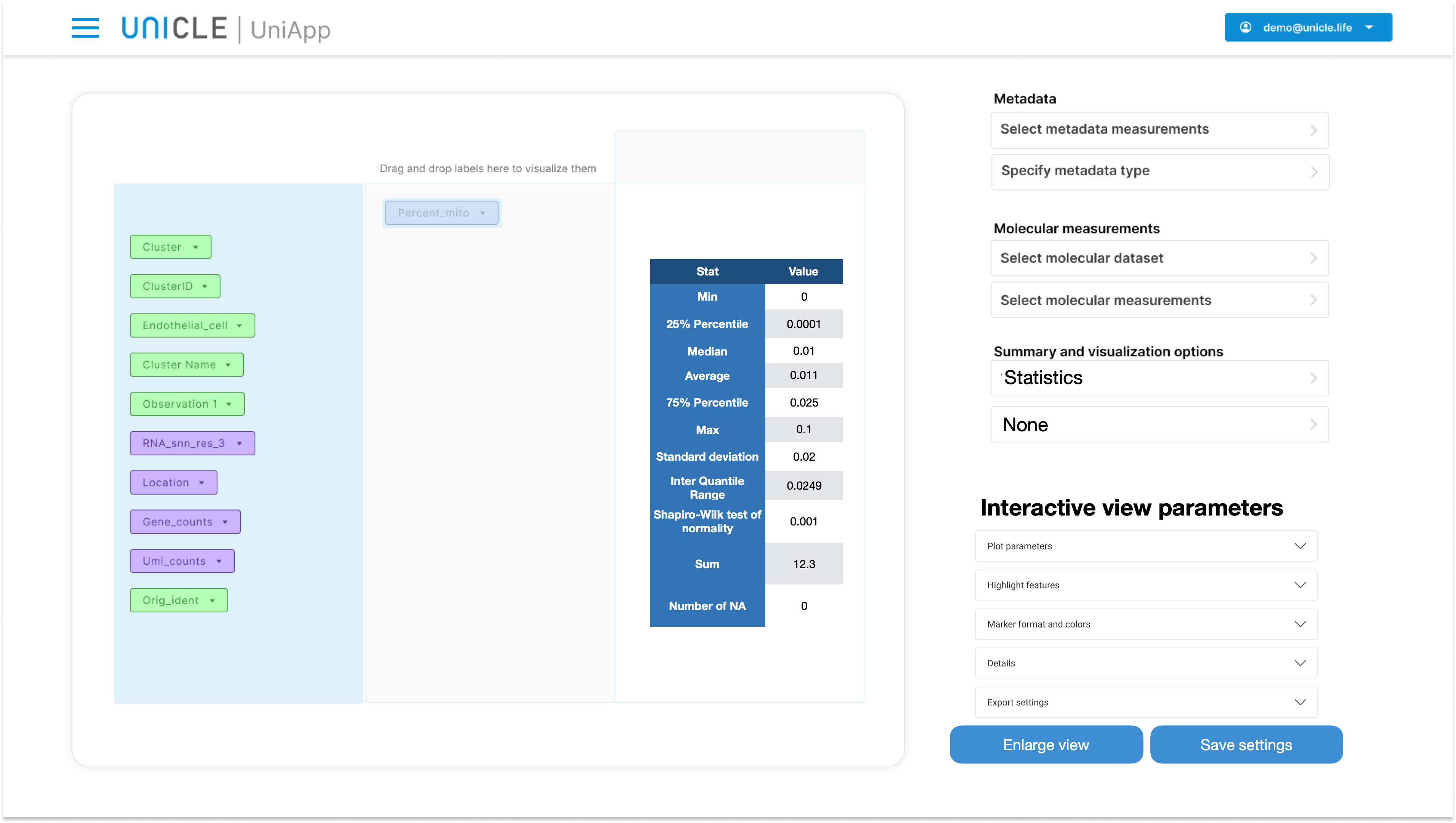`Important: any time a table or a plot is visualized in the Pivot Table, Panel five is modified in two ways:1) The button "Save settings" appears below the table/plot. This button allows to open a new view where the table or plot can be saved within a Track.2) A number of menus appear under the title "Interactive view parameters". These menus allow to interactively modify the table / plot contained in Panel3) The button "Enlarge view" appears as well. This button can be used for enlarging Panel 4, while Panel 1, 2 and 3 are folded away.`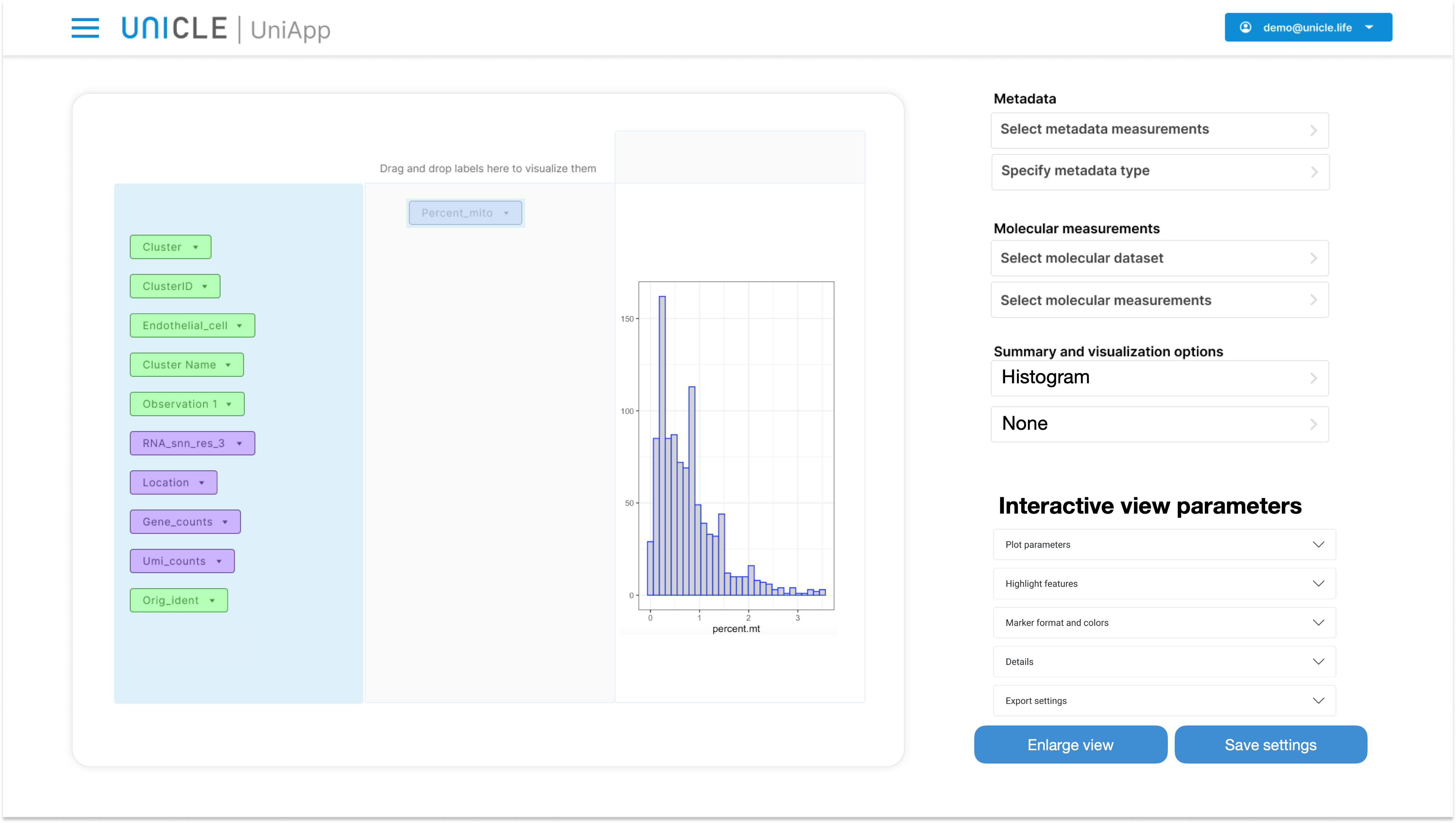Pressing the "Enlarge view" button will magnify Panel 4: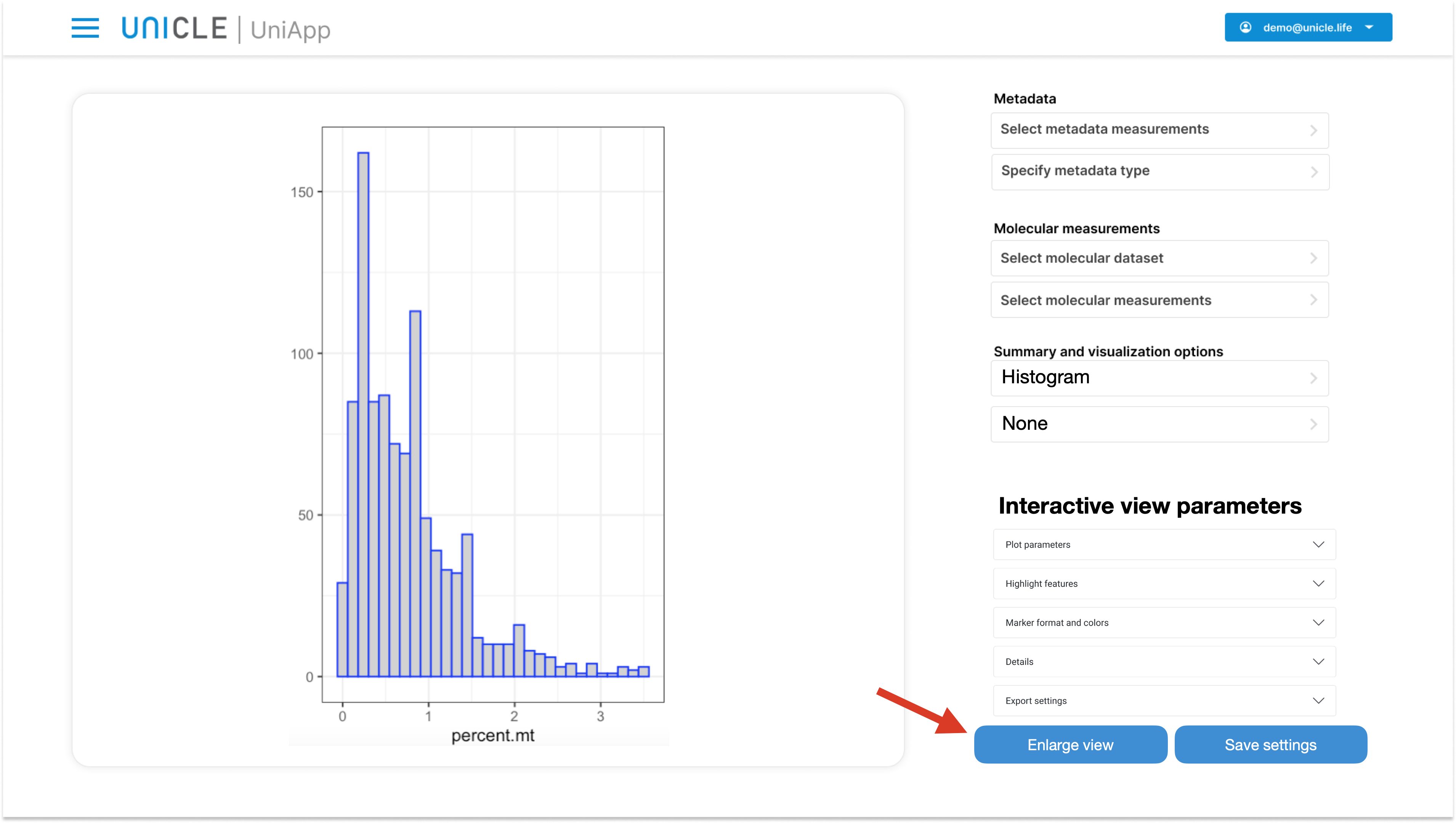Finally, selecting the density plot will land this visualization: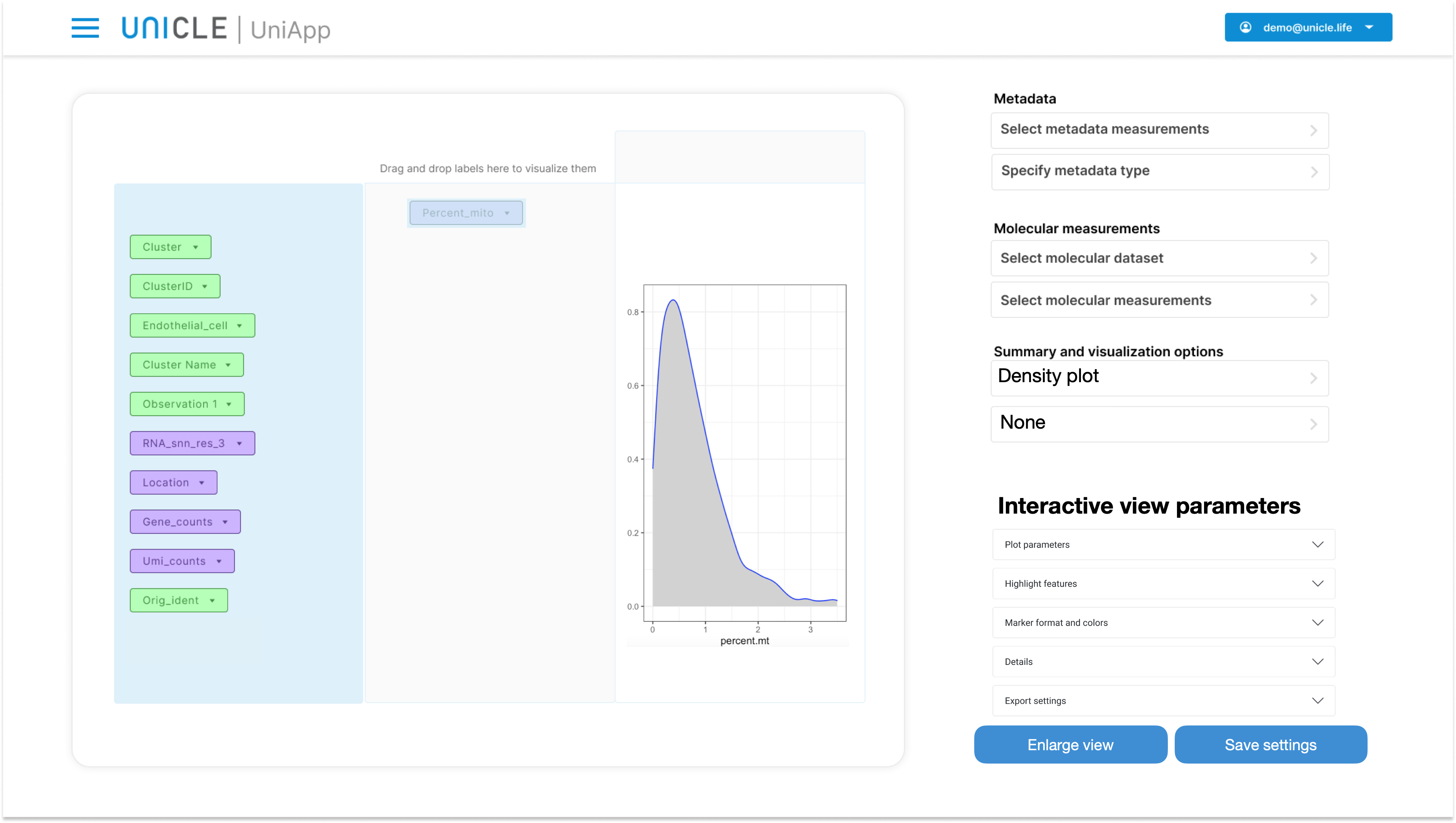`Important: once a numerical variable is dropped in Panel 2 or 3, no other variable can be dropped in the same panel. `

### 2.4.2 Visualizing a single categorical variable

When a categorical variable is dropped in Panel 3 or Panel 5, there will be two different visualization options, while no aggregation method will be available:

1. Statistics
2. Bar plot

Choosing the Statistics visualization will display a table of statistics, which includes counts and frequency for each category. The least frequent and the most frequent (mode) category are also marked.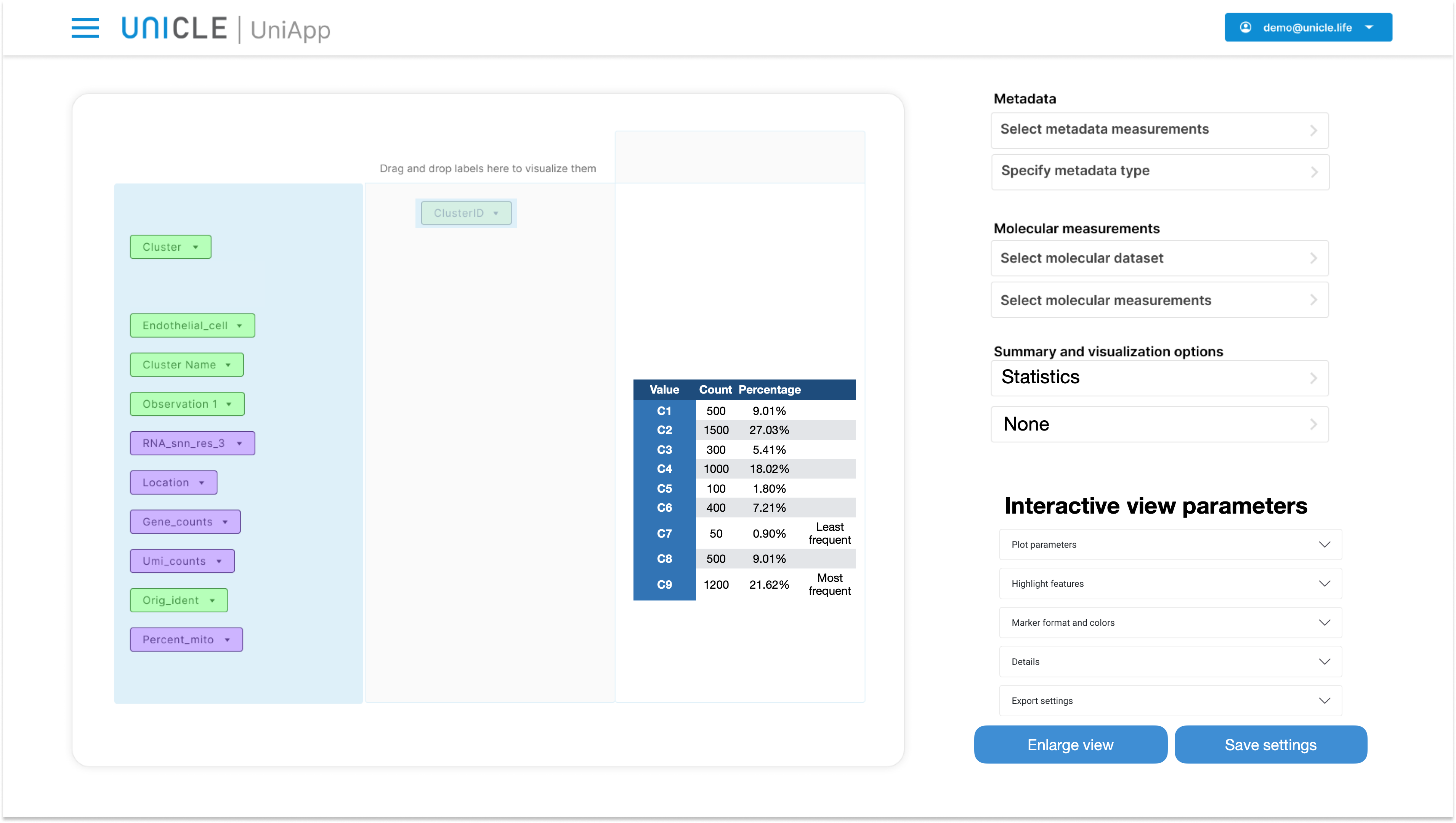The bar plot visualization is as follow, where the summary is replaced by a graph: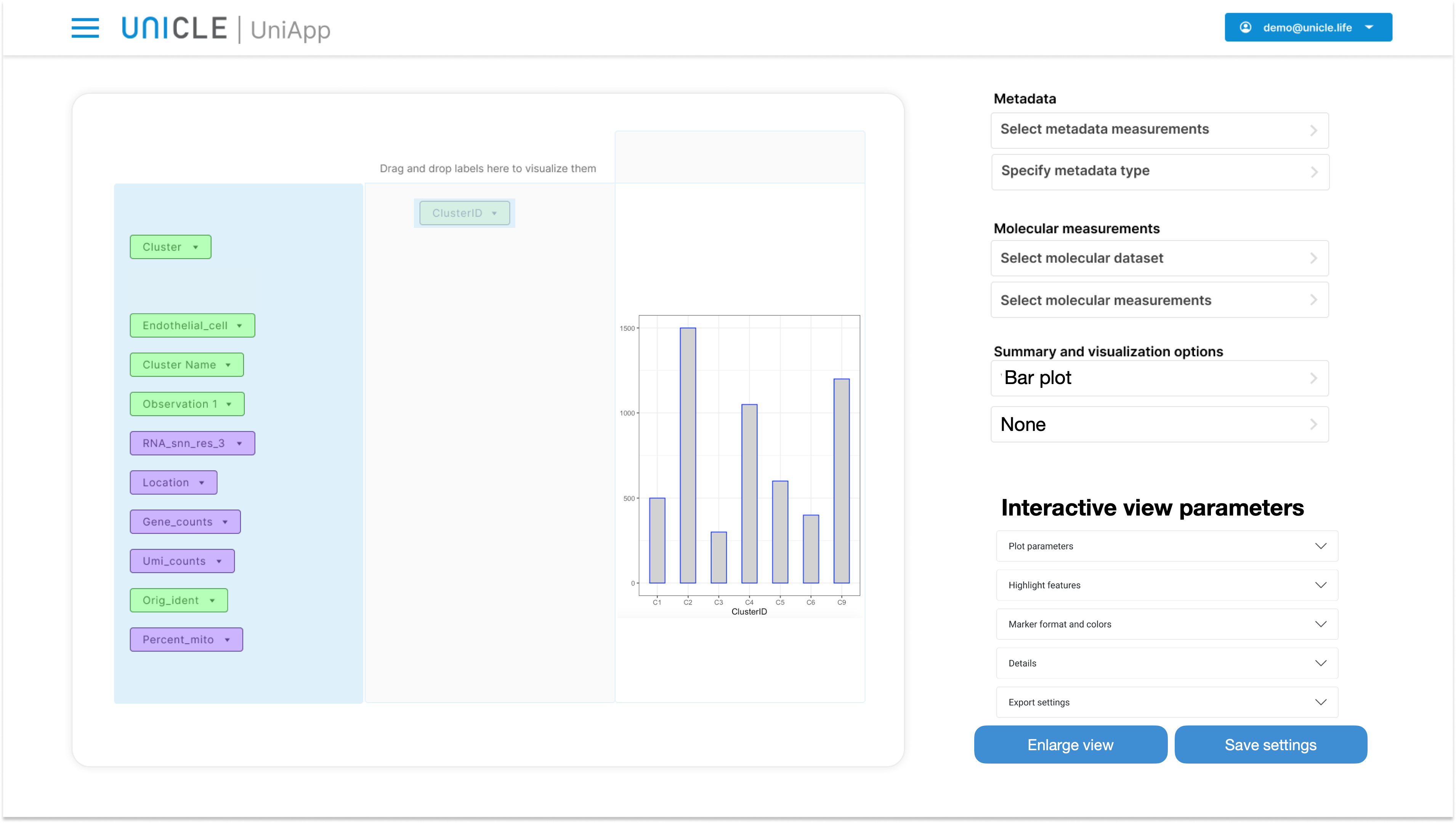Contrarily to what happens with numerical variables, it is possible to drop more than a categorical variable in Panel 2 or 3. See section "7 Combining several categorical variables in a single axis" for details.

`Categorical variables with more than 100 values by default will generate a warning and the user will be asked to confirm that they want to proceed with the visualization. This is because a large number of categories usually leads to plots difficult to interpret. `

## 2.5 Visualizing variables pairs

In order to visualize the joint distribution of two variables, one of them must be dropped from Panel 1 to Panel 2, and one of them from Panel 1 to Panel 3. The available visualizations and data aggregation approaches depend upon the chosen variables types. The possible alternatives are described below.

### 2.5.1 Visualizing two numerical variables

Dropping one numerical variable in Panel 2 and one in Panel 3 will allow to investigate their joint distribution. The "Summary type" dropdown menu will be disabled, while the available visualization types will be:

• Statistics: correlation analysis
• Statistics: linear model
• Scatterplot
• Density plot

The "Statistics: correlation analysis" visualization is reported below. The pivot table reports the Pearson, Spearman and Tau correlation coefficients, along with their corresponding p-values. both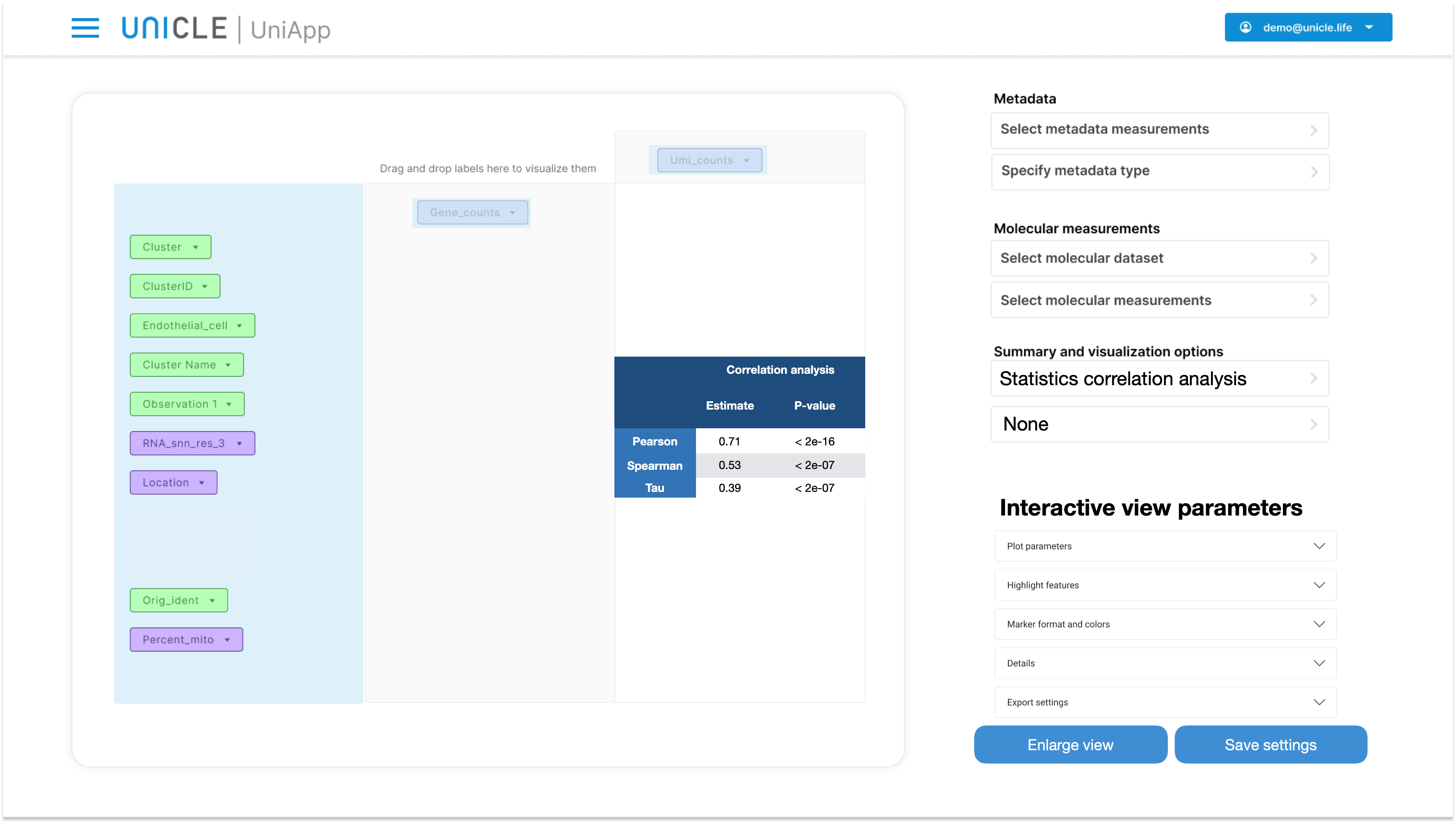Chosing "Statistics: linear model" will fit a linear model between the two variables.Particulary, the variable dropped in Panel 3 acts as independent variable (x-axis) and the variable dropped in Panel 2 as dependent variable (y-axis).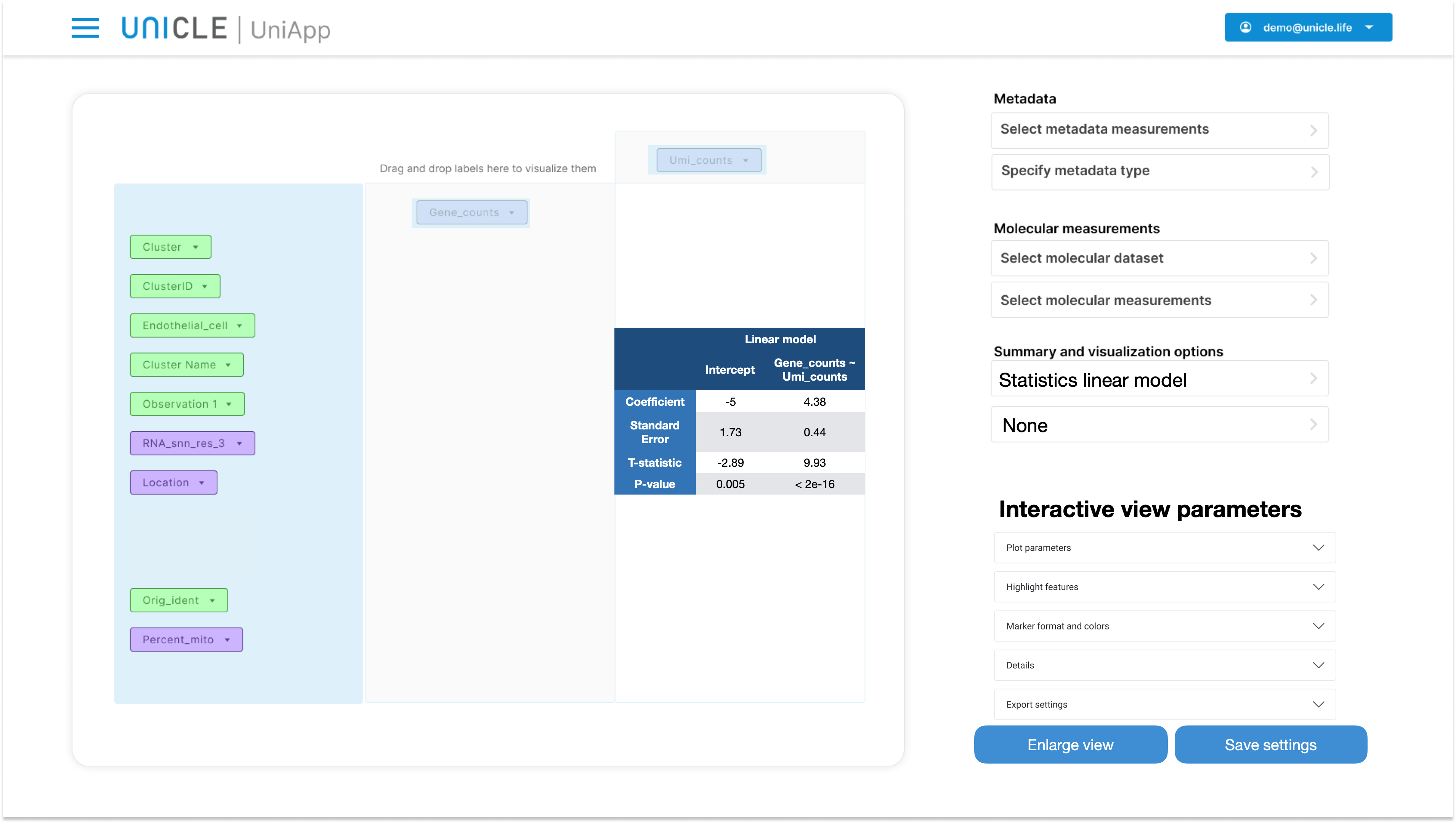Passing to the scatterplot visualization will produce the following: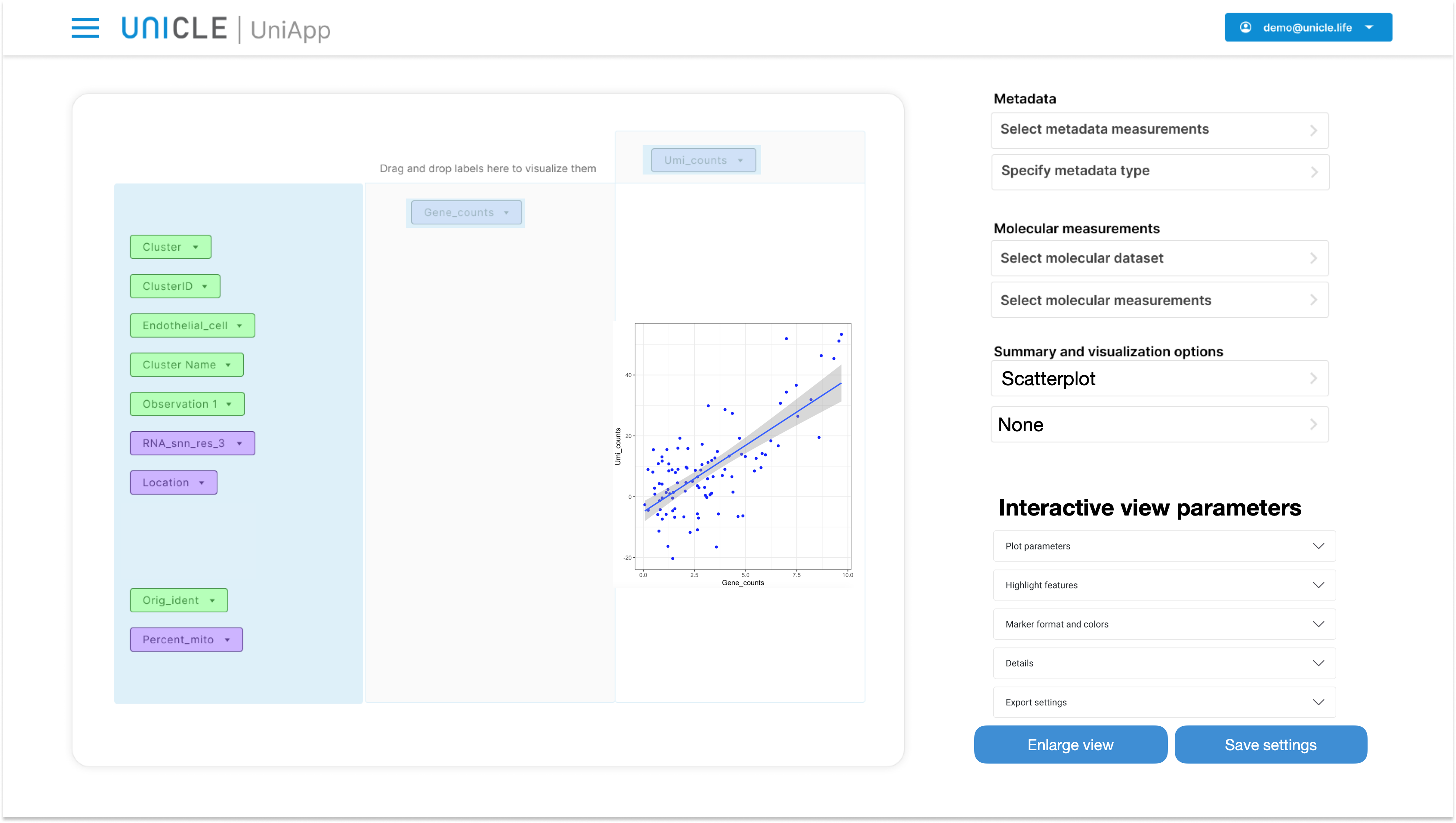The density visualization simply replaces the scatter plot with a 2D density plot: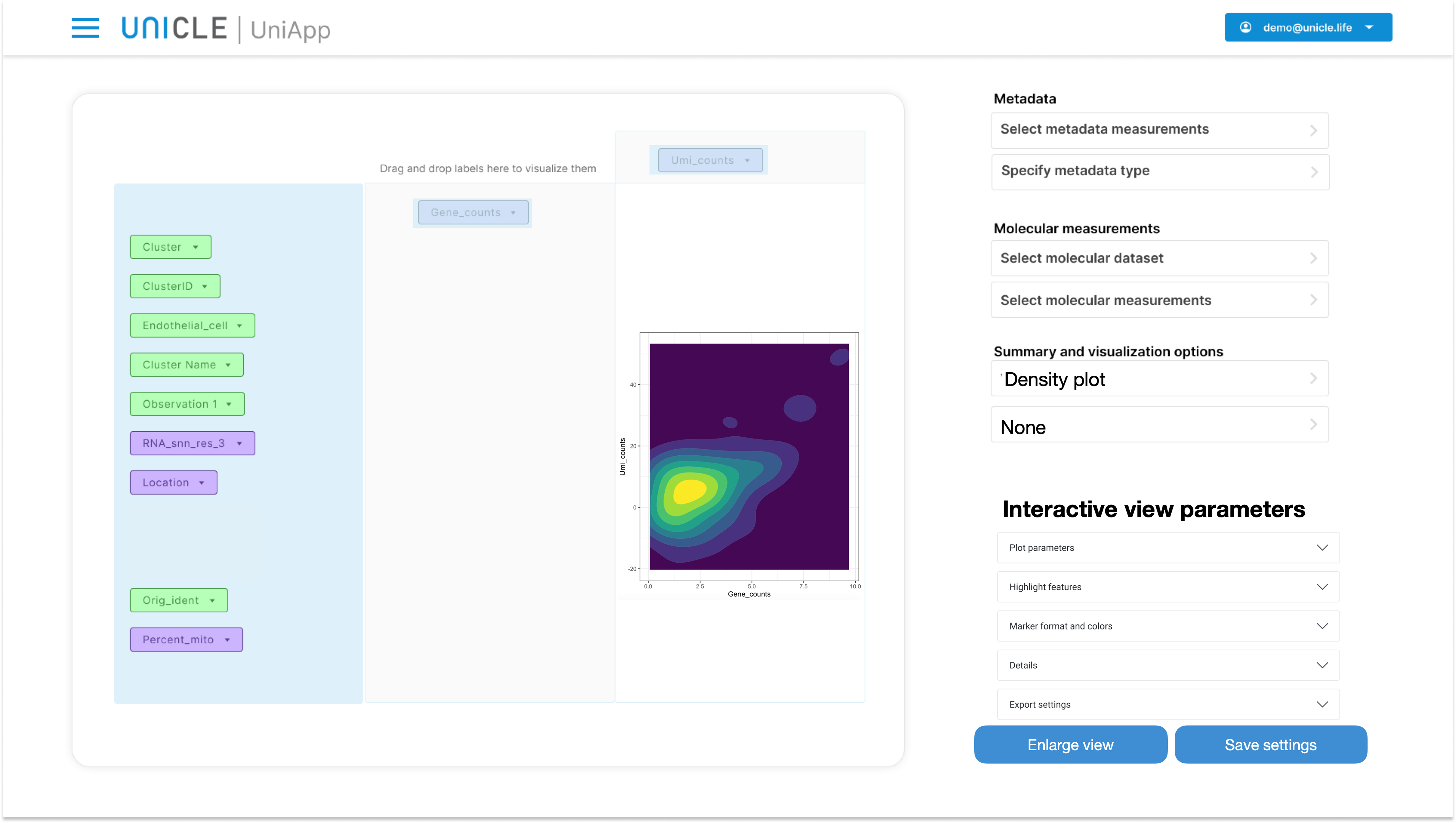### 2.5.2 Visualizing two categorical variables

Numerous visualization types are available for representing the joint distribution of two categorical variables

1. Statistics: contingency table
2. Statistics: association test
3. Bar plots
4. Heatmap
5. Sankey plot
6. Mosaic plot

Furthermore, two summary types are also available:

1. Counts
2. Frequencies

Let's start with visualizing descriptive statistics, i.e., contingency tables. This generates a table with row corresponding to the values of the categorical variable dropped in Panel2, while the column correspond to the values of the variable in Panel 3. If summary type is set to count, each cell of the table reports the number of samples corresponding to the respective row and column. If the summary type is set to frequencies, than each cell reports the proportion of samples falling in its combination of values. Marginal counts and frequencies are also displayed.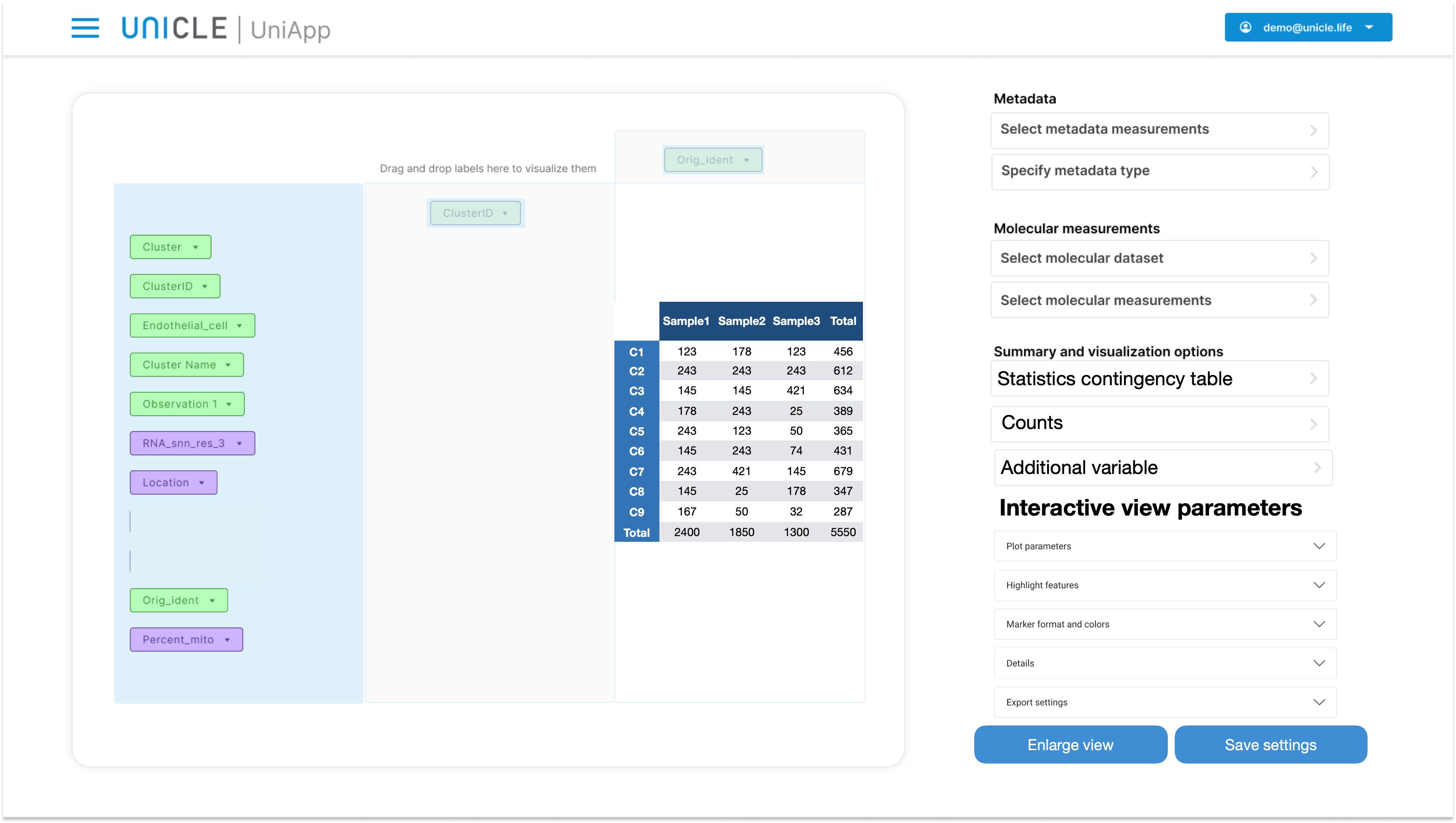The next visualization type presents the results of the chi squared test assessing whether the there is any association between the two chosen variables. For 2 x 2 tables the Fisher exact test is used instead of chi squared.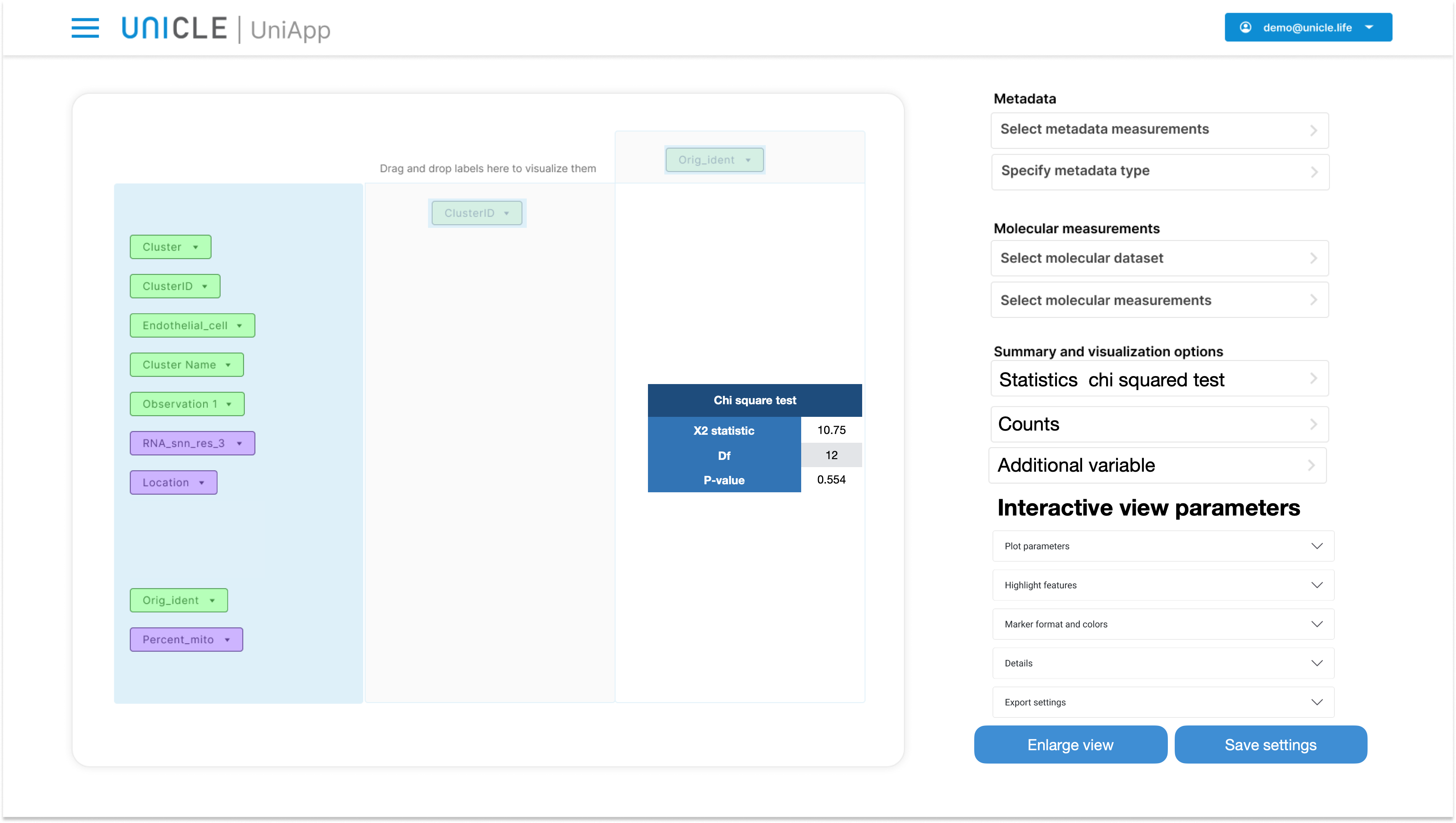The Bar plot visualization provides a quick overview of the data. Depending on the choice in the summary type dropdown meny, this height of the bars will correspond either to the counts or to the frequencies of each category combination. Note that the variable dropped in Panel 3 will be placed on the x-axis of the plot, while the variable in Panel2 will indicate the color of the bars.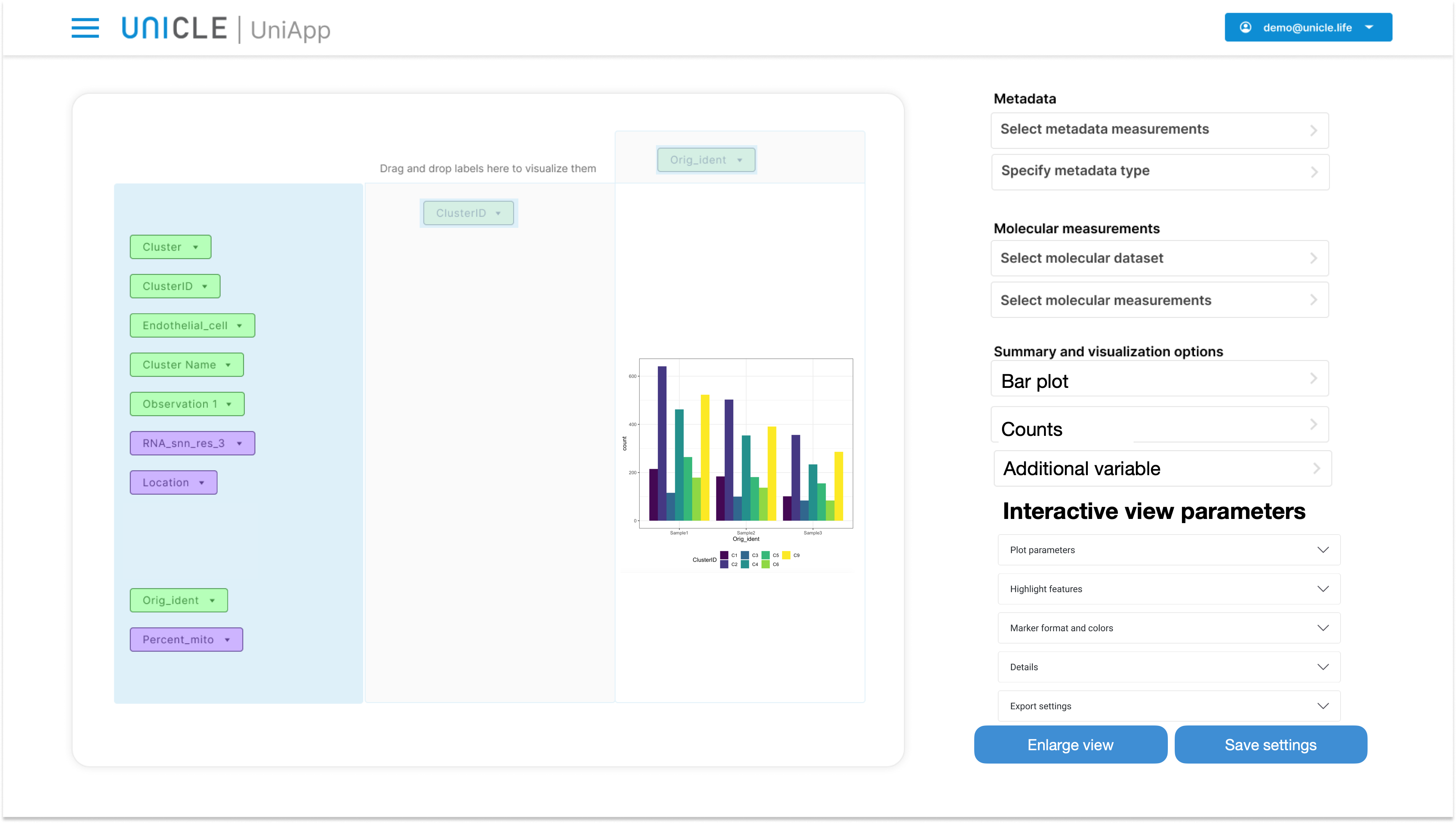Heatmaps are direct translation of table into figures; a heatmap is organized as a table, with colors indicating how many samples (counts) or what proportion (frequencies) of samples are present in each heatmap cell.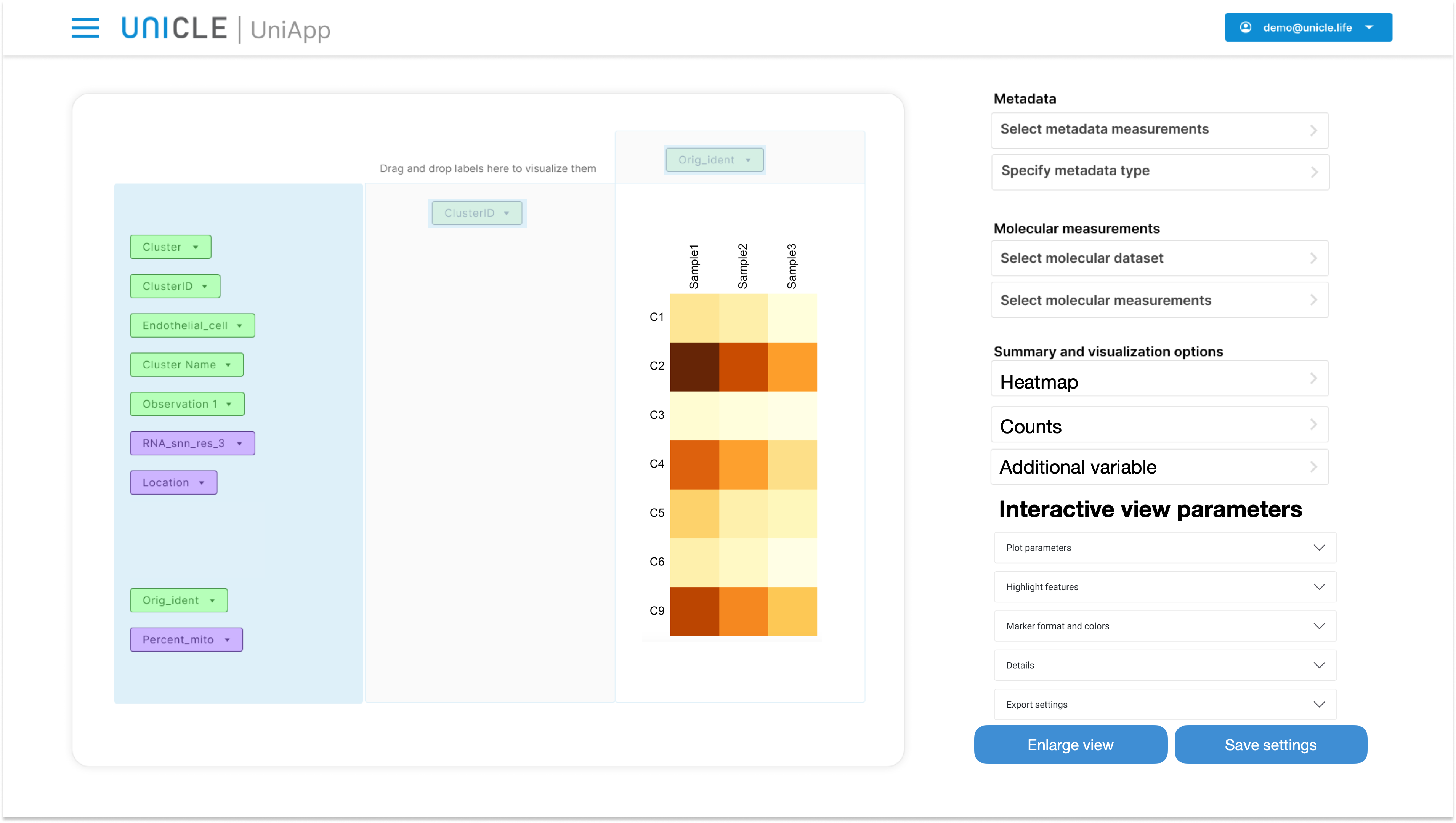Sankey plots are one additiona tool to graphically represent how samples are distributed across two variables. Only counts can be visualized in Sankey plots, not frequencies.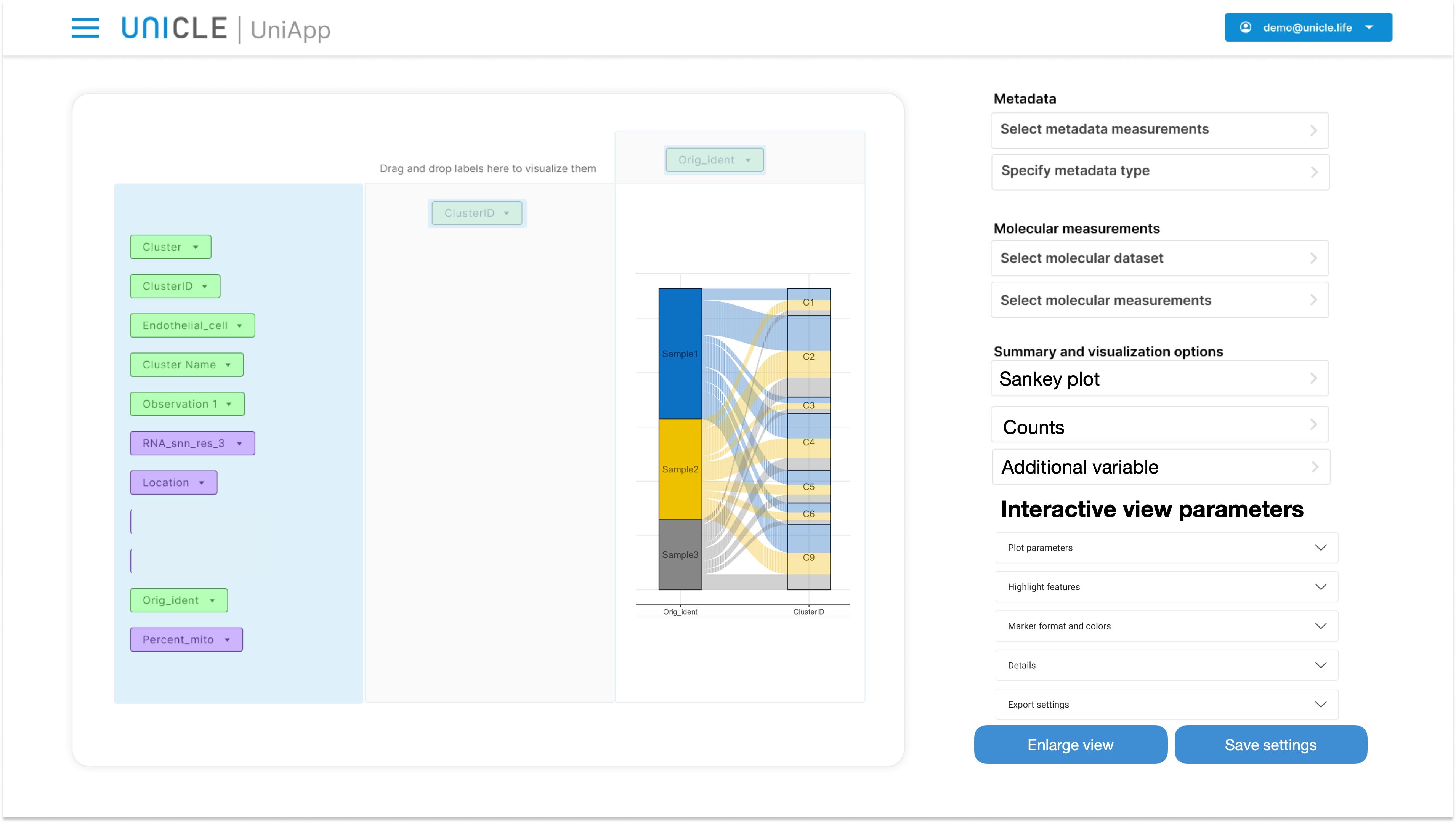Finally, Mosaic plots are a graphically appealing variation with respect to the classical barplots. Only frequencies can be represented in this type of plots.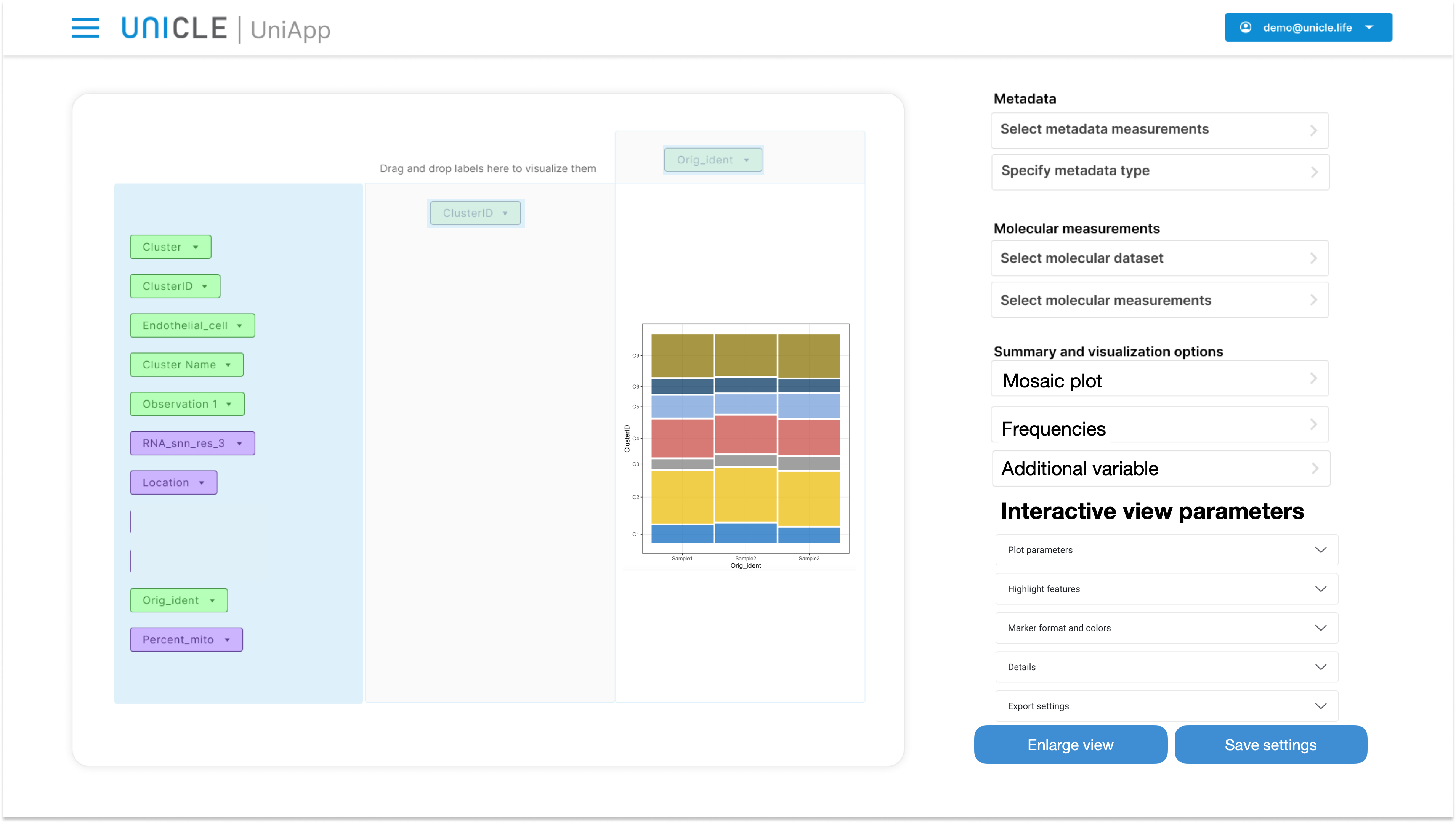### 2.5.3 Visualizing one categorical variable and a numerical variable

When a numerical variable is selected along side a categorical one, the available sample is divided in subgroups according to the categorical variable and the distribution of the numerical variable can be studied within each subgroup. The available visualization types for this case are the following:

1. Statistics: descriptive
2. Statistics: anova test
3. Bar plot
4. Heatmap
5. Box plot

The descriptive statistics include:

1. Average
2. 25% Quantile
3. Median
4. 75% Quantile
5. Maximum
6. Standard deviation
7. Interquantile range
8. Sum
9. Number of missing values

The summary type menu is disabled when the "Statistics: descriptive" visualization type is active.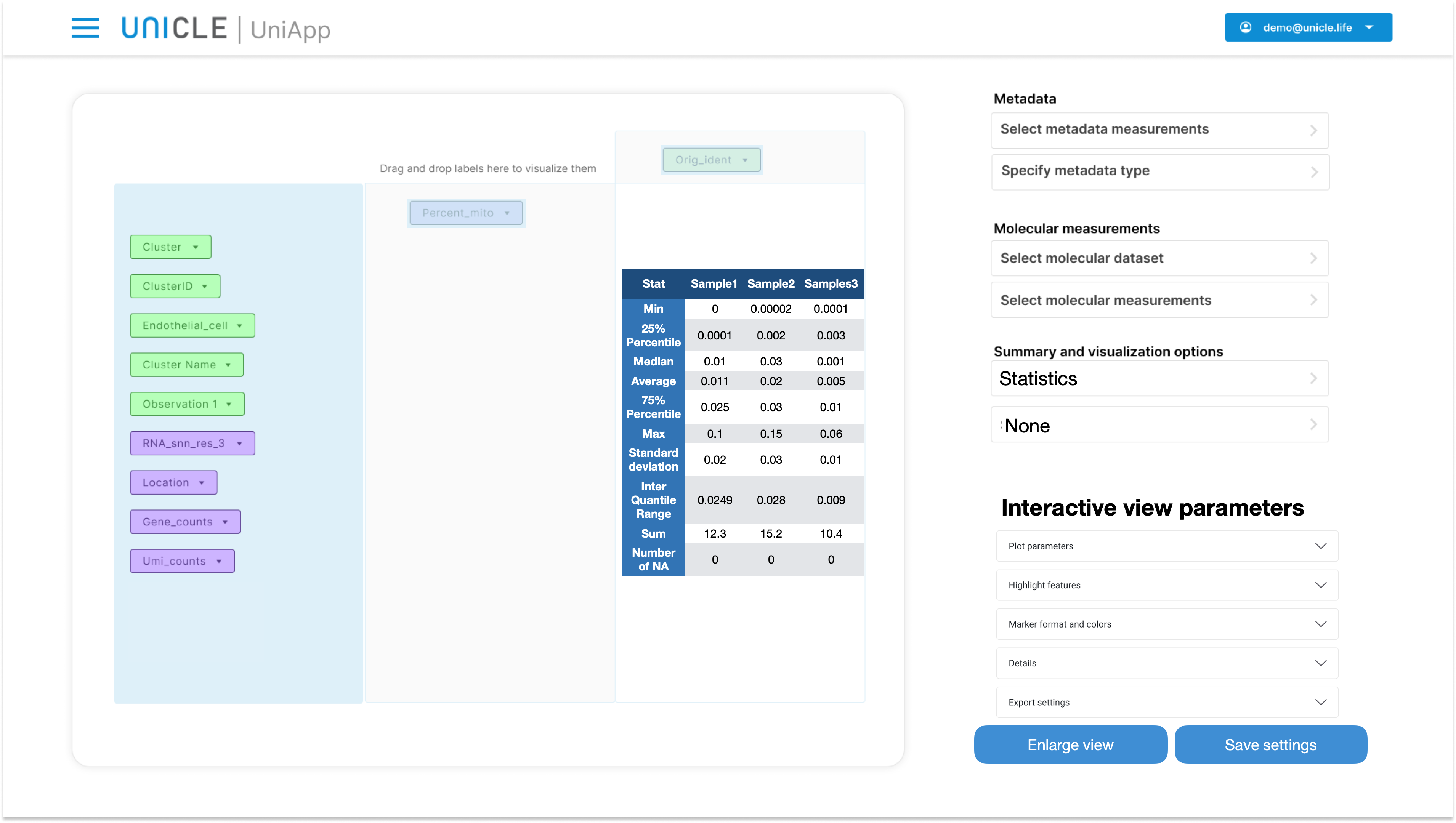The "Statistics: anova test" visualization reports the results of an ANOVA test, or a t-test if the categorical variable is binary: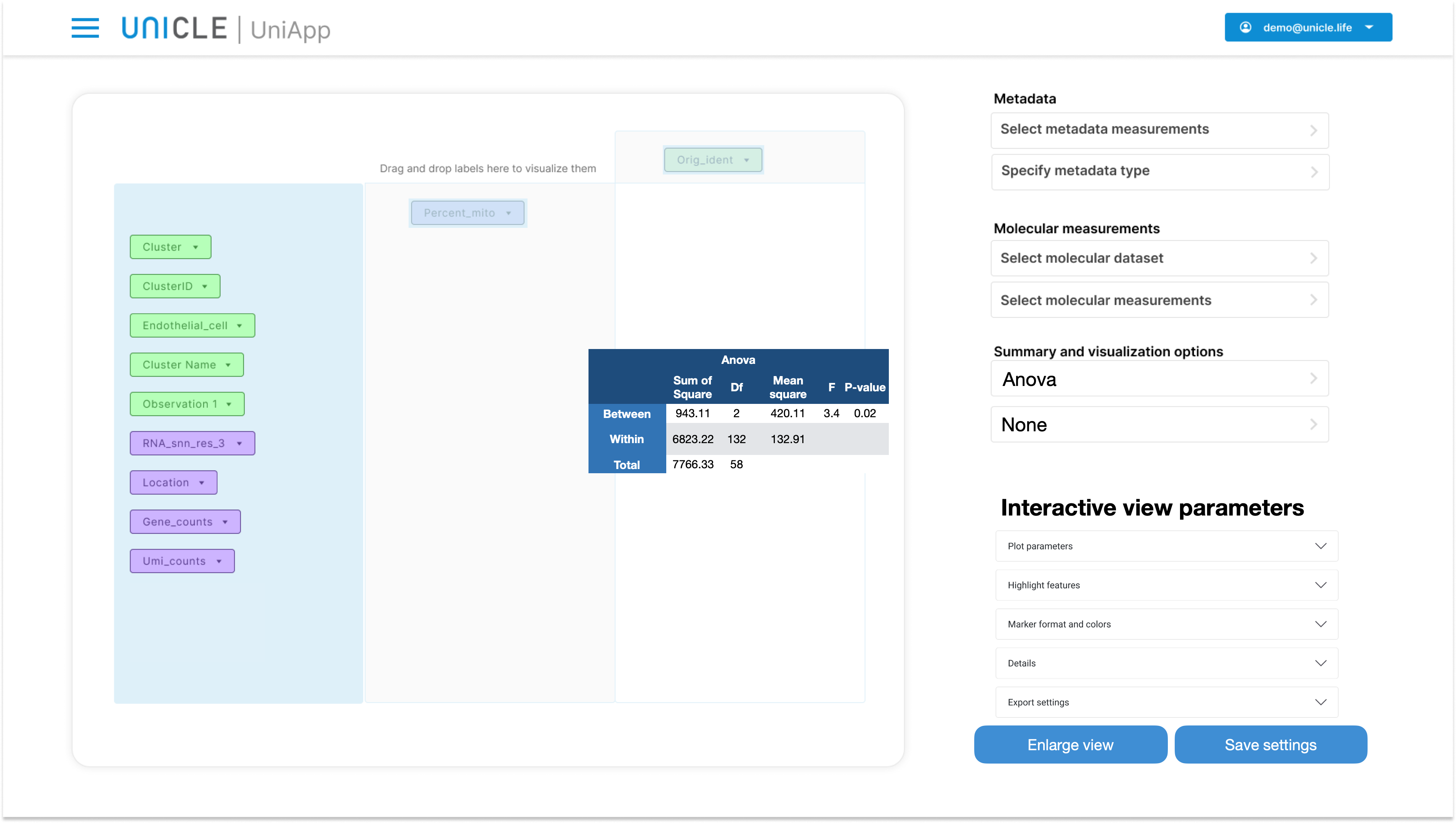Barplots provides one more opportunity for exploring the distribution of the numerical variable within each category of the categorical variable. When the users select the bar plot visualization type, they must also select one of the options out of the Summary types. The eight of the bars then reflect the chosen summary type. For example, this is the plot resulting from selecting bar plot as visualization type and the average as summary type: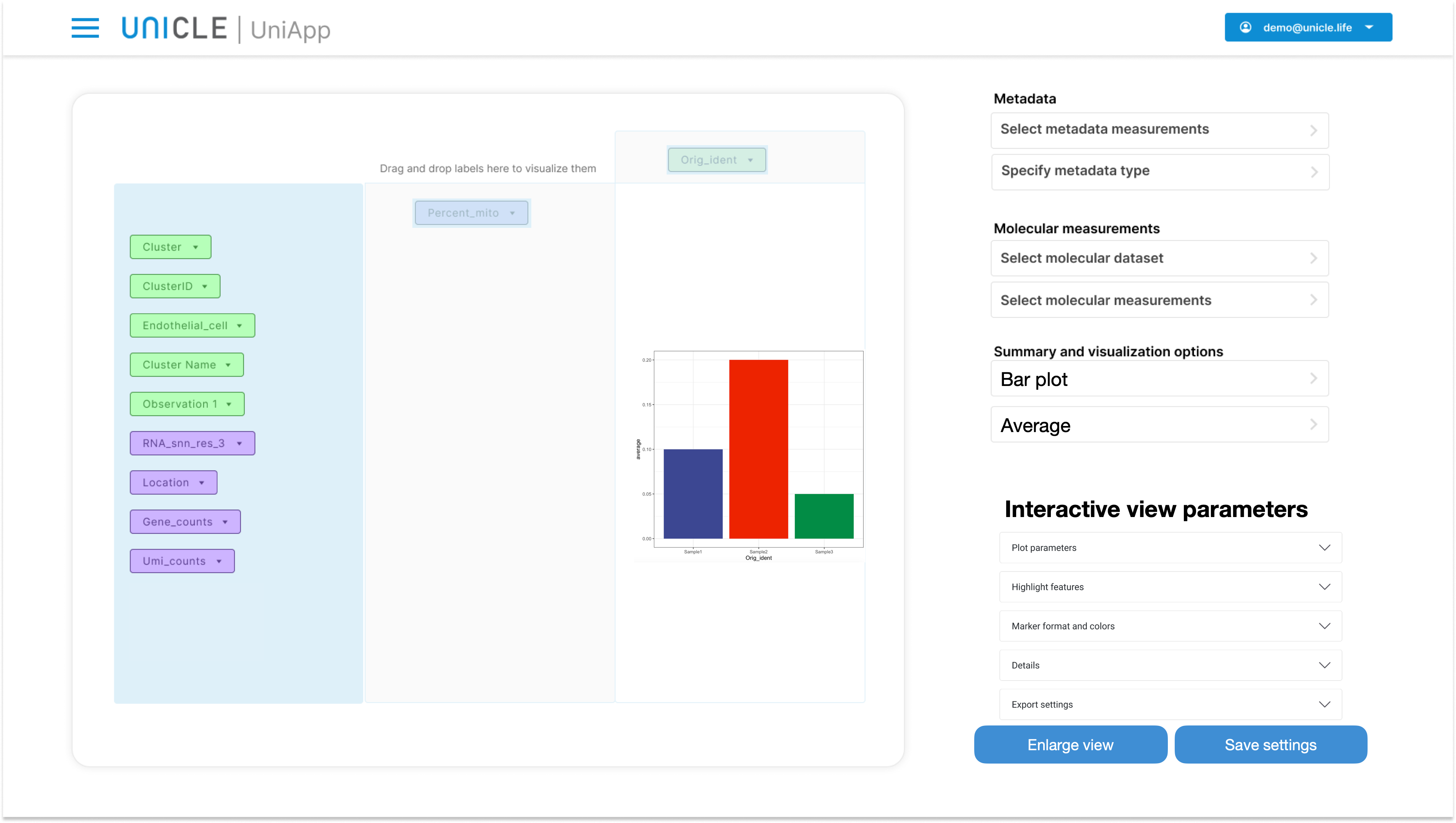The following plot was instead obtained by selecting "standard deviation" in the summary type dropdown menu: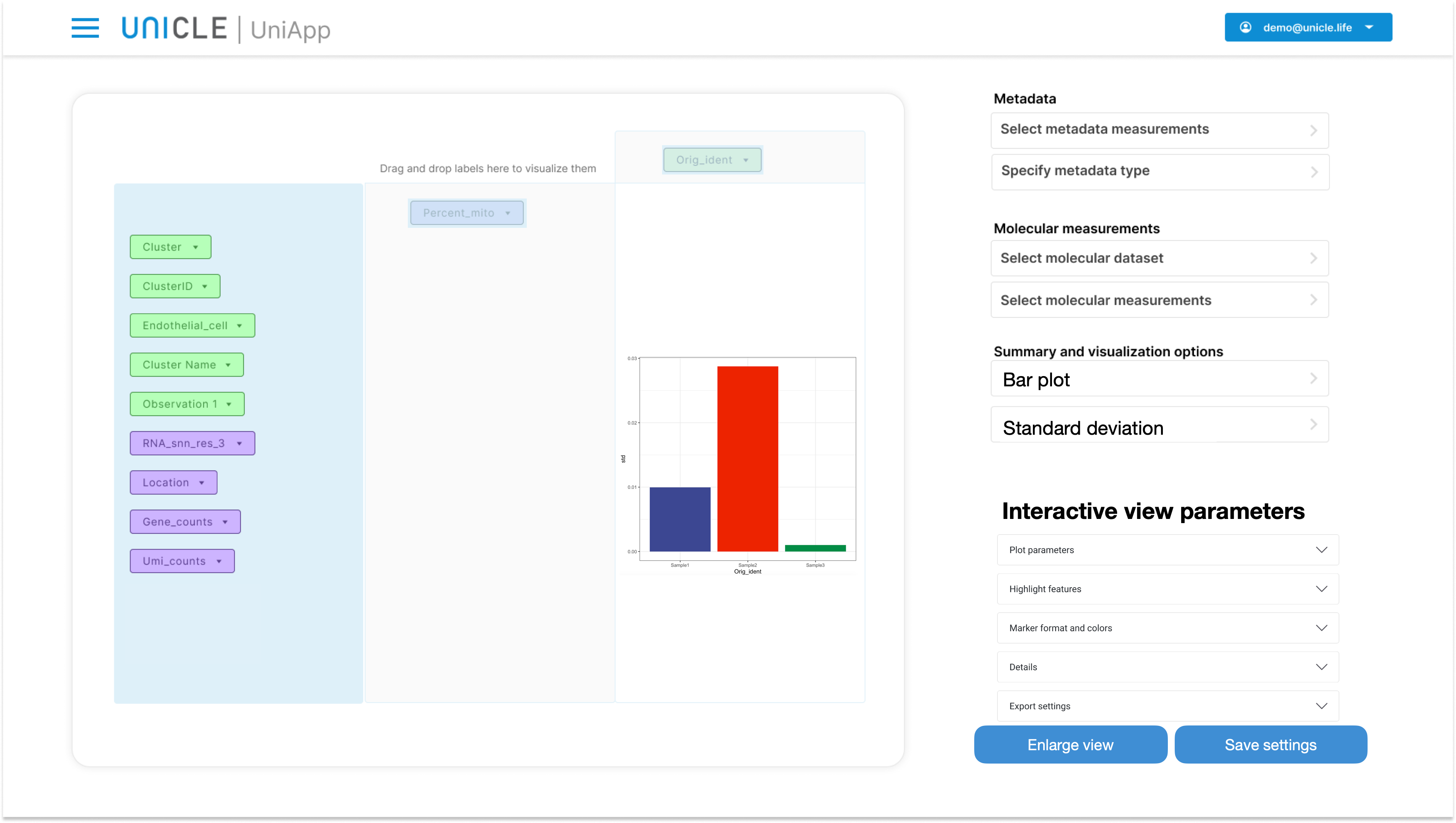Heatmaps work in a way that is identical to the bar plots approach, using shade of color rather than height for comparing different summary statistics across categories.

More interestingly, box plots and violin plots provide a bird-eye view on several aspects of the distribution of the continuous variable. The selection of the summary type is deactivated when box plots are shown. Violin plots can be constructed using the interactive view parameters.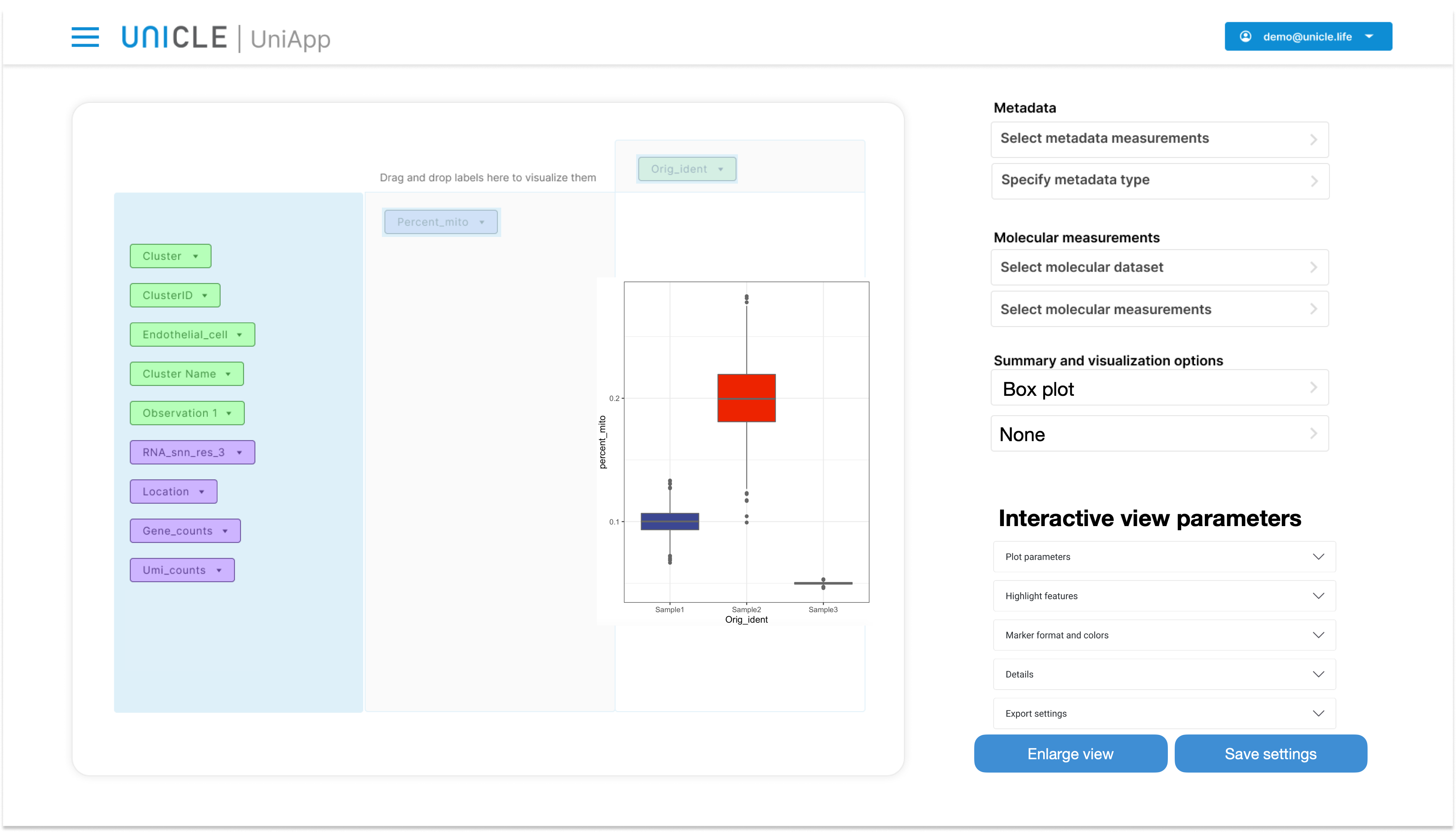## 2.6 Visualizing two categorical and one numerical variables

When two categorical variables are selected (see section 5.2 above), a fourth drop down menu appear in Panel 5.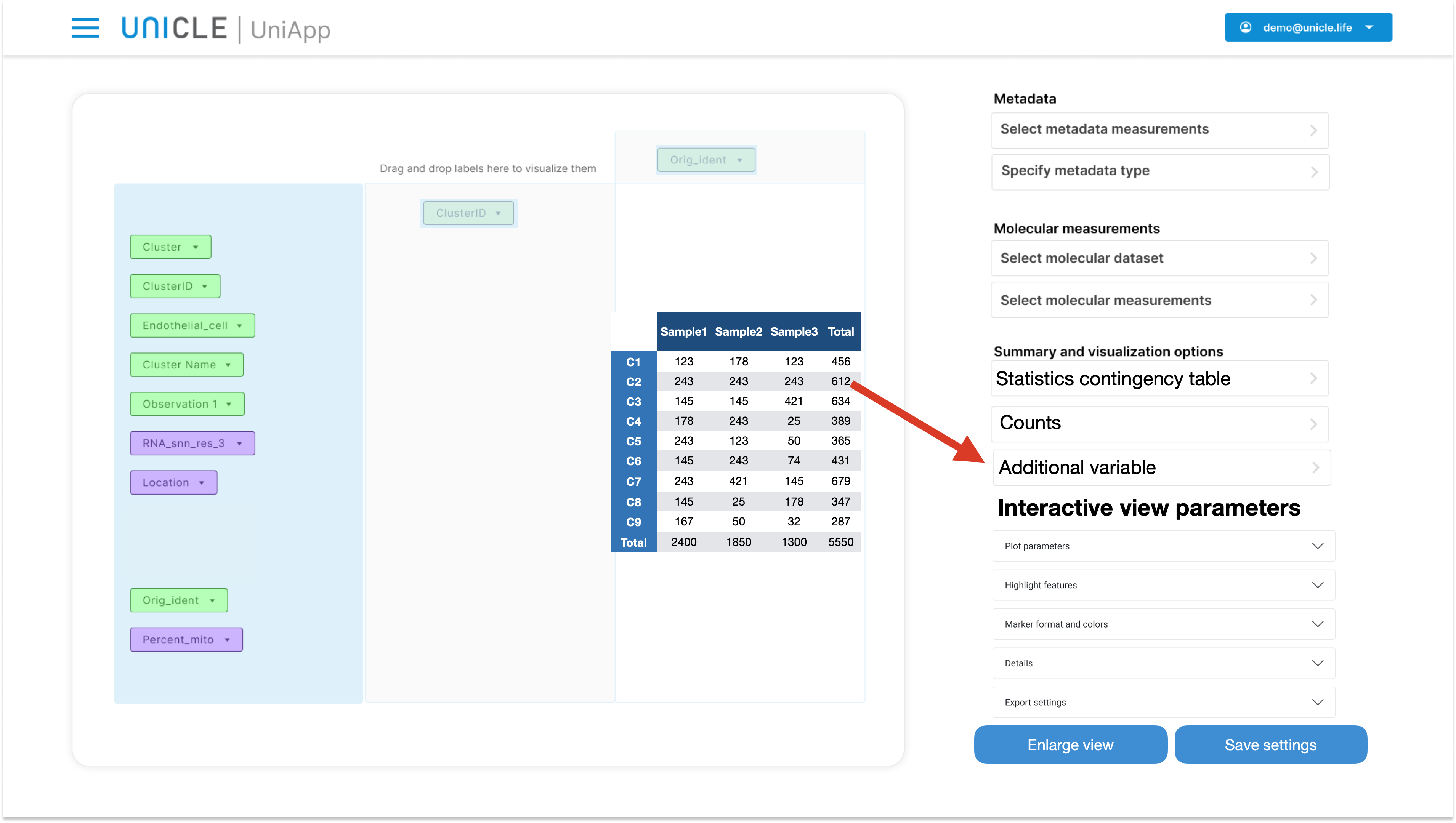This menu allows to select one numerical variable among the ones contained in Panel 1. Once this variable is selected, the cell of the pivot table will start visualizing summary statistics computed on the selected variable. For example, in the figure below, "Percent_mito" was selected as additional variable, "Average" was selected as Summary type, and thus the cells of the Pivot Table report the average of Percent_mito within each subgroup defined by the two chosen categorical variables.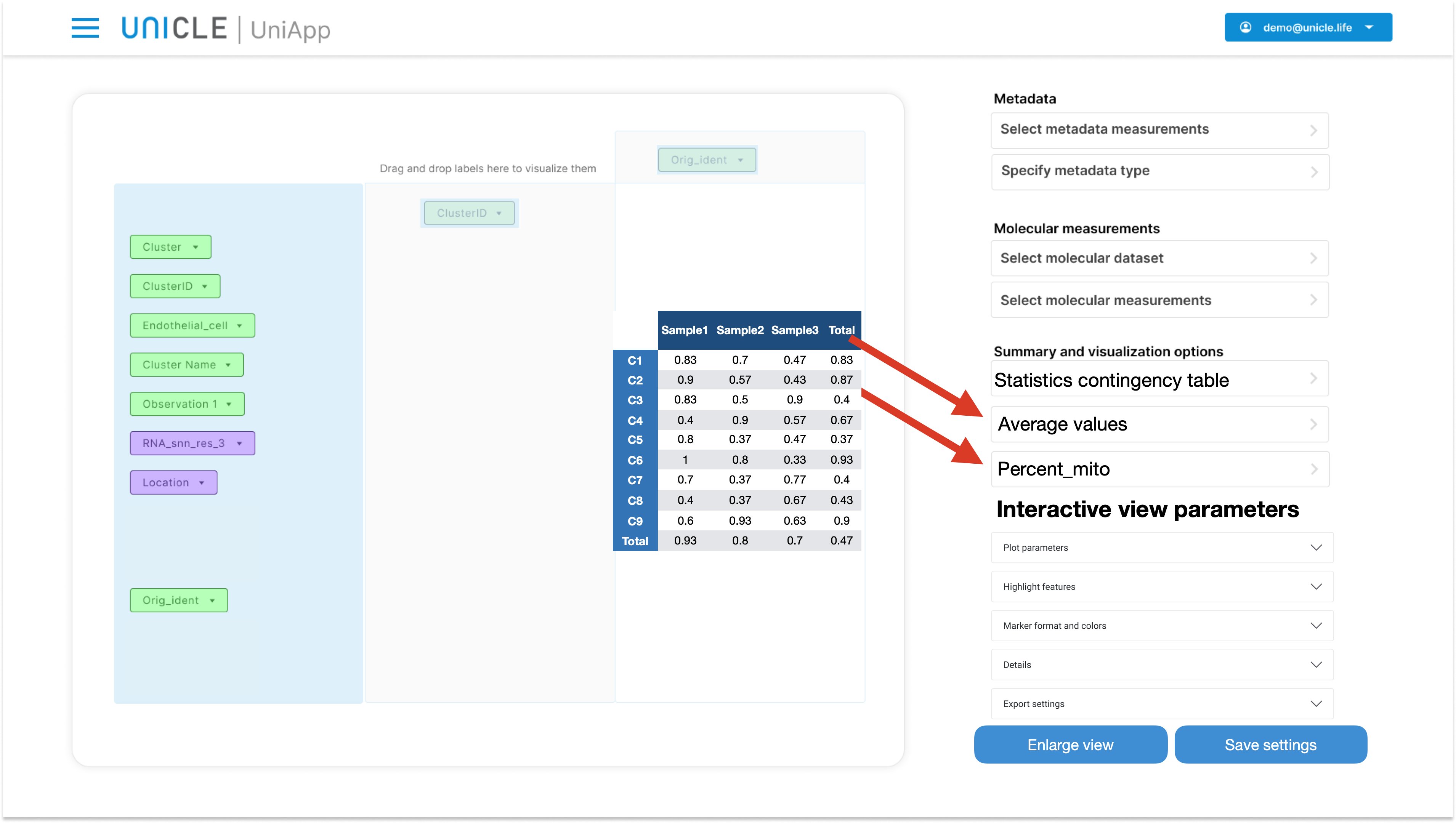Summary statistics available for the additional continuous variable are the same used in section "5.3 Visualizing one categorical variable and a numerical variable". Also the available visualization types are the same:

1. Statistics: descriptive
2. Statistics: anova test
3. Bar plot
4. Heatmap
5. Box plot

The Anova and Heatmap visualizations operates as in section 5.3. Bar plots and box plots needs to be slightly modified in order to represent all three variables. For bar plots, one categorical variable dictates the x-axis, the other regulate the color of the bars, and the chosen summary statistic from the numerical variable decides the height of the bars.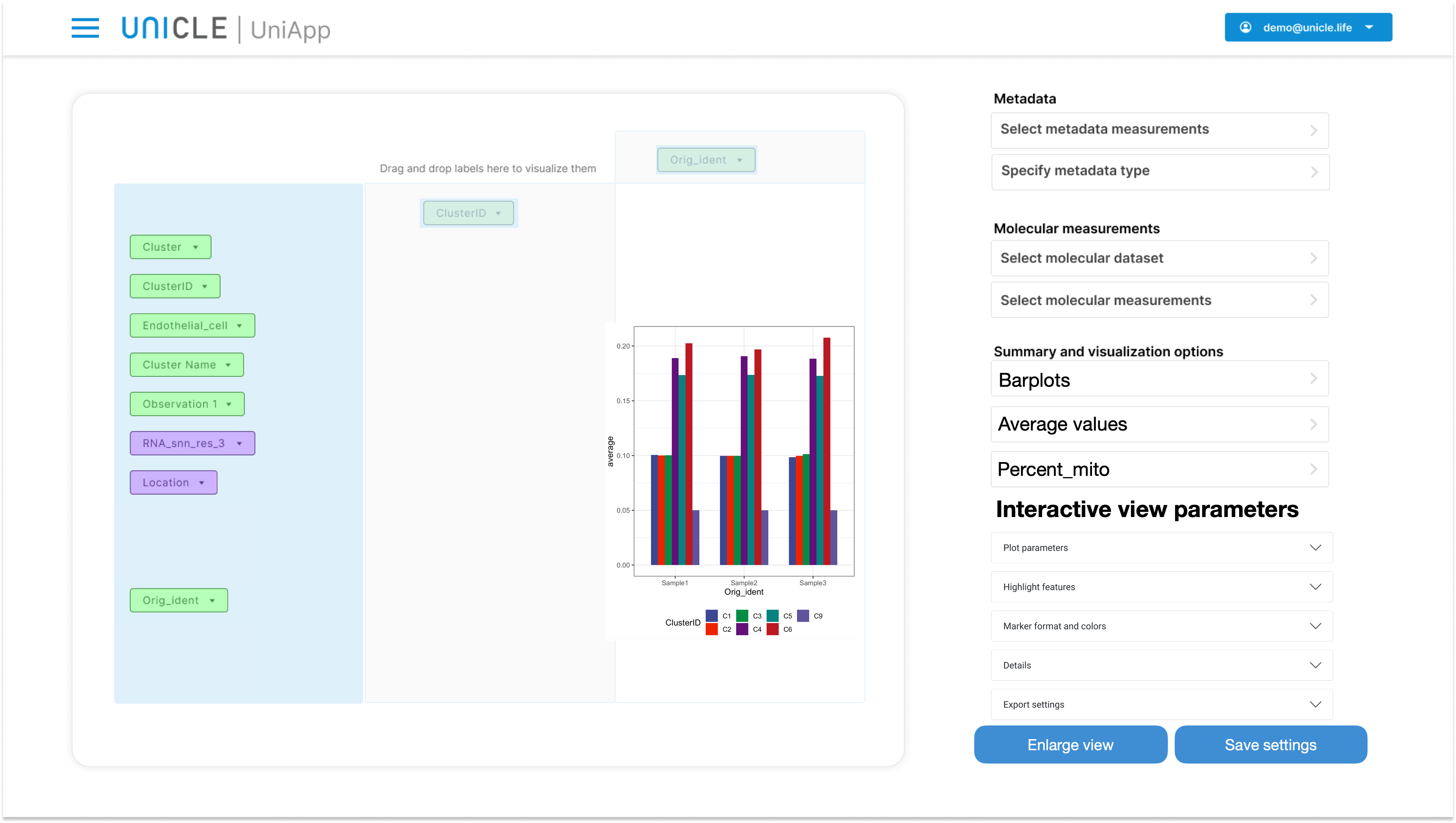Box plots works in a similar way, however no summary statistics needs to be selected: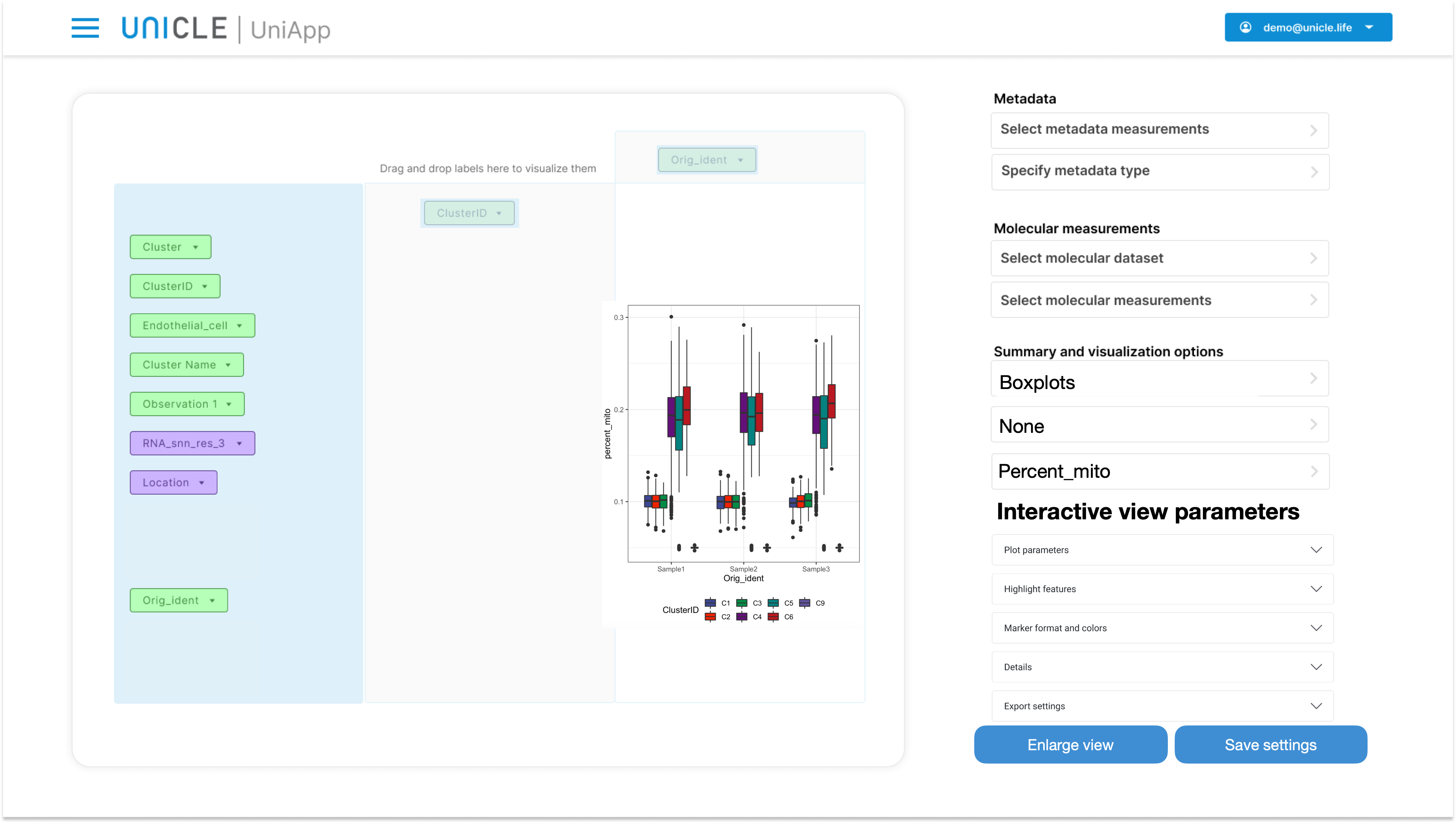## 2.7 Combining several categorical variables in a single axis

Two or more categorical variable can be simultaneosuly being included in a single axis, by dragging both of them either in Panel 2 or Panel 3. In this case, the categories of these variables are combined so that to create a single list of categories. For example, ClusterID and Orig_ident are combined together in Panel 2: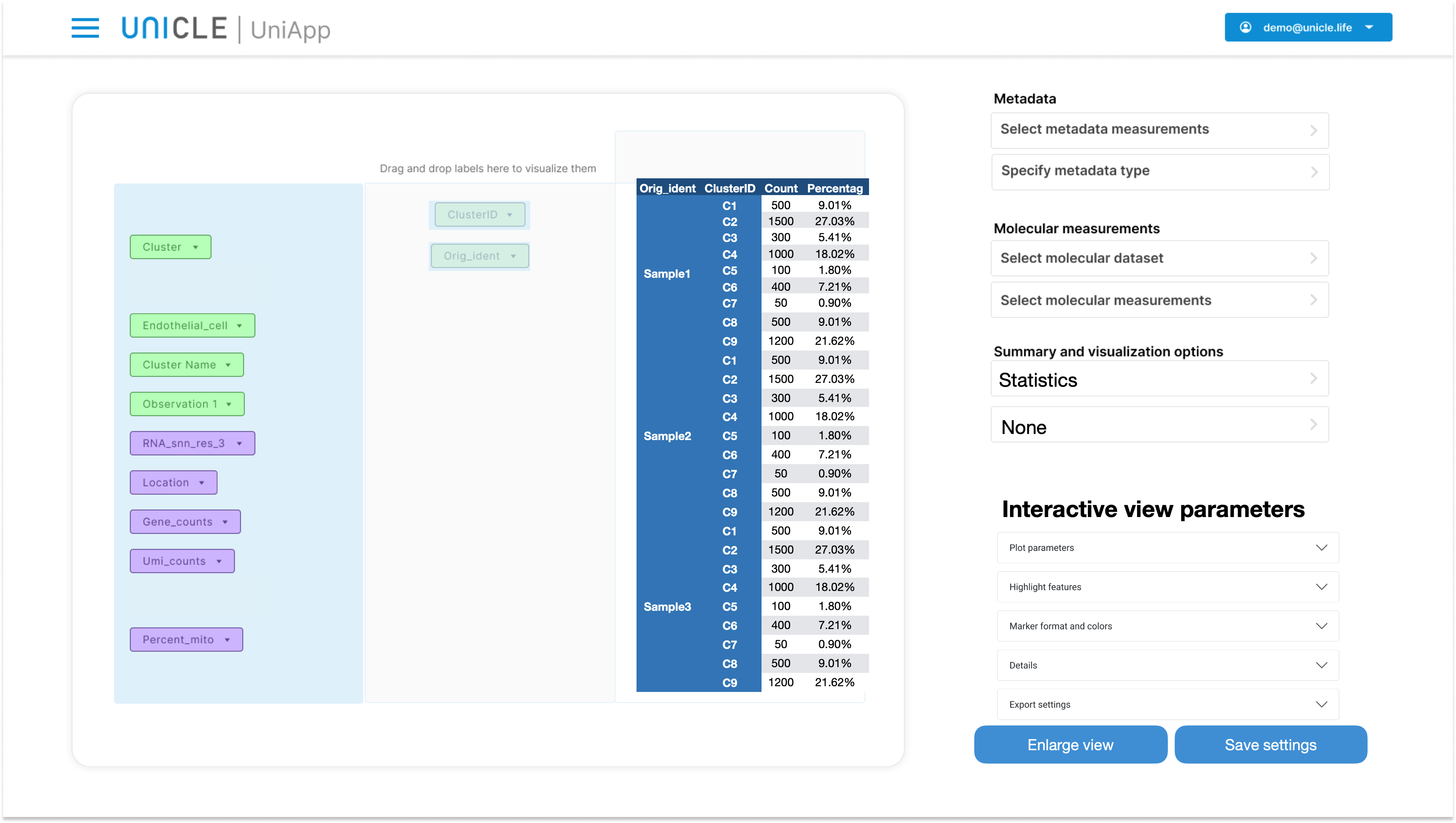This Pivot Table can now be analyzed as if a single categorical variable was in Panel 2. Other variables can be added to Panel 3 as well for more in depth explorations.

## 2.8 Visualizing date variables in a Gantt chart

The Pivot table in the Management analytics screen is able to visulize date variables as a Gantt chart. A Gantt chart is a special type of bar chart that illustrates a project schedule. The tasks of project are listed on the y-axis while the start and expected due date of the task are plotted on the x-axis. To create a Gantt chart select the "Gantt chart" display option. Then drag the task variable in the y-axis and the start and due dates of the task in the y-axis. Addiotionally, dragging in addiotional categorical variables will color-code the bars of the Gannt chart. For example, adding the "Assignee" variable will show which task is assigned to which person.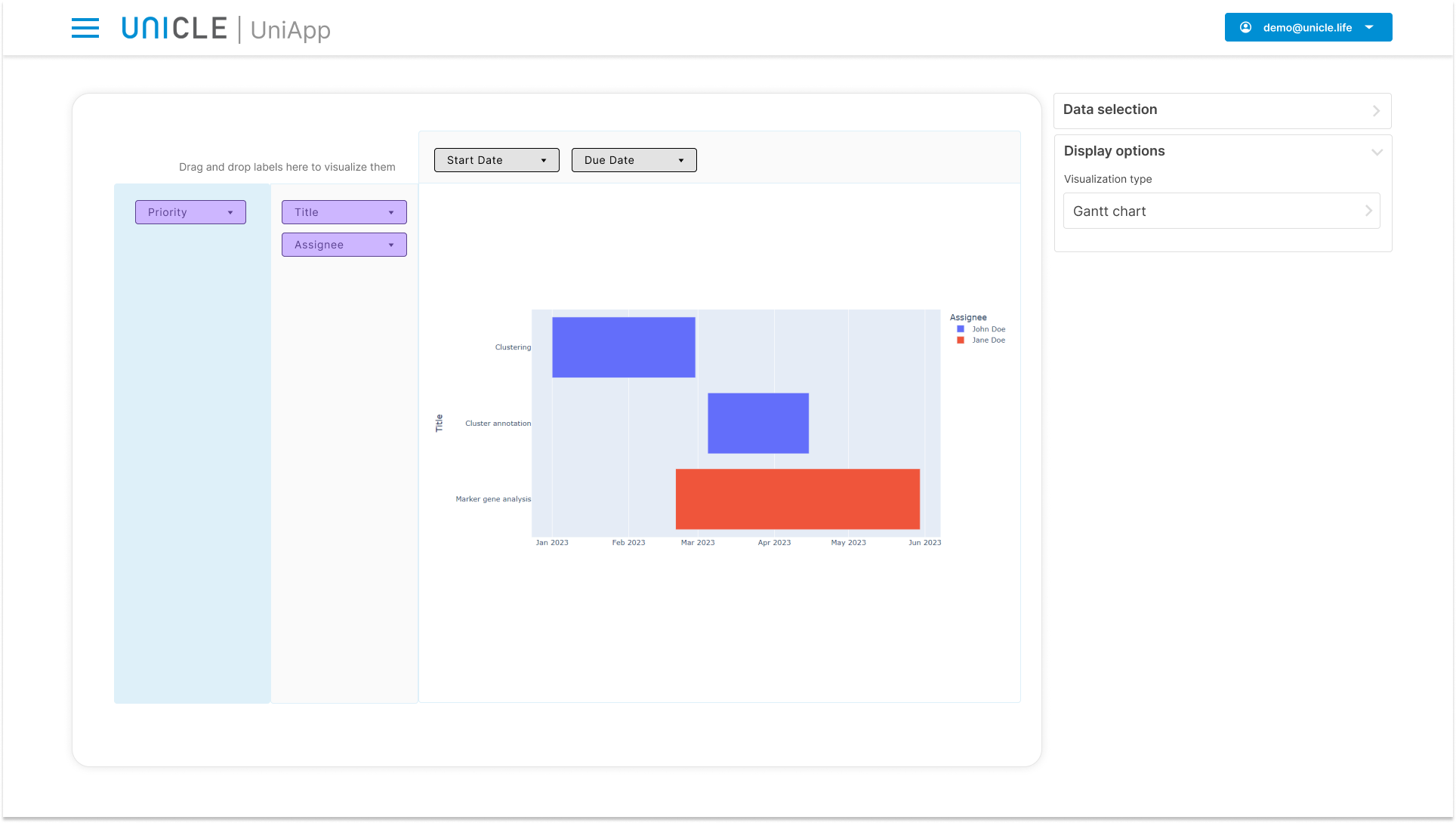# 3. Saving plots

From Save output tab you can save the plot you have currently set up as a new track element. Frst add the plot name and desctiption, then click on the Save button. The plot should now appear in the analysis track.